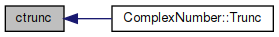amath  1.8.5 Simple command line calculator
mathi.h File Reference

Complex numbers math library. More...

This graph shows which files directly or indirectly include this file: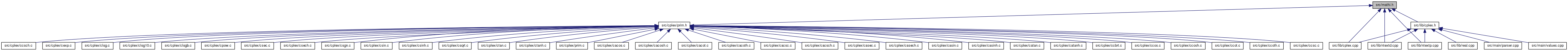Go to the source code of this file.

union  complex

## Functions

double csgn (complex z)
Complex signum. More...

double cabs (complex z)
Absolute value of complex number. More...

double creal (complex z)
Real part of complex number. More...

double cimag (complex z)
Imaginary part of complex number. More...

complex cpack (double x, double y)
Pack two real numbers into a complex number. More...

complex cadd (complex a, complex z)
Addition of two complex numbers. More...

complex csub (complex a, complex z)
Subtraction of two complex numbers. More...

complex cmul (complex a, complex z)
Multiplication of two complex numbers. More...

complex cdiv (complex a, complex z)
Division of two complex numbers. More...

complex cpow (complex x, complex z)
Complex number raised to a power. More...

complex cceil (complex z)
Ceiling value of complex number. More...

complex cfloor (complex z)
Floor value of complex number. More...

complex ctrunc (complex z)
Truncated value of complex number. More...

complex cround (complex z)
Division of two complex numbers. More...

complex creci (complex z)
Reciprocal value of complex number. More...

complex conj (complex z)

complex cexp (complex z)
Returns e to the power of a complex number. More...

complex csqrt (complex z)
Square root of complex number. More...

complex ccbrt (complex z)
Cube root of complex number. More...

complex clog (complex z)
Natural logarithm of a complex number. More...

complex clogb (complex z)
Base 2 logarithmic value of complex number. More...

complex clog10 (complex z)
Base 10 logarithmic value of complex number. More...

complex ccos (complex z)
Cosine of complex number. More...

complex csin (complex z)
Sine of a complex number. More...

complex ctan (complex z)
Tangent of a complex number. More...

complex csec (complex z)
Secant of a complex number. More...

complex ccsc (complex z)
Cosecant of a complex number. More...

complex ccot (complex z)
Cotangent of a complex number. More...

complex cacos (complex z)
Inverse cosine of complex number. More...

complex casin (complex z)
Inverse sine of complex number. More...

complex catan (complex z)
Inverse tangent of complex number. More...

complex casec (complex z)
Inverse secant expressed using complex logarithms: More...

complex cacsc (complex z)
Inverse cosecant of complex number. More...

complex cacot (complex z)
Inverse cotangent of complex number. More...

complex ccosh (complex z)
Hyperbolic cosine of a complex number. More...

complex csinh (complex z)
Hyperbolic sine of a complex number. More...

complex ctanh (complex z)
Hyperbolic tangent of a complex number. More...

complex csech (complex z)
Hyperbolic secant of a complex number. More...

complex ccsch (complex z)
Hyperbolic secant of a complex number. More...

complex ccoth (complex z)
Hyperbolic cotangent of a complex number. More...

complex cacosh (complex z)
Inverse hyperbolic cosine of complex number. More...

complex casinh (complex z)
Inverse hyperbolic sine of complex number. More...

complex catanh (complex z)
Inverse hyperbolic tangent of complex number. More...

complex casech (complex z)
Inverse hyperbolic secant of complex numbers. More...

complex cacsch (complex z)
Inverse hyperbolic cosecant of complex number. More...

complex cacoth (complex z)
Inverse hyperbolic cotangent of complex number. More...

## Detailed Description

Complex numbers math library.

Mostly as specified in [IEEE Std 1003.1, 2013 Edition]: http://pubs.opengroup.org/onlinepubs/9699919799/basedefs/complex.h.html

Definition in file mathi.h.

## ◆ cabs()

 double cabs ( complex z )

Absolute value of complex number.

Definition at line 54 of file prim.c.

References cimag(), creal(), and hypot().

Referenced by ComplexNumber::Absolute(), clog(), cpow(), and csqrt().

55 {
56  return hypot(creal(z), cimag(z));
57 }
double cimag(complex z)
Imaginary part of complex number.
Definition: prim.c:46
double creal(complex z)
Real part of complex number.
Definition: prim.c:38
double hypot(double x, double y)
hypot
Definition: hypot.c:81
Here is the call graph for this function: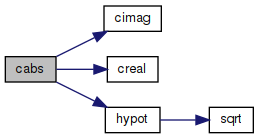Here is the caller graph for this function: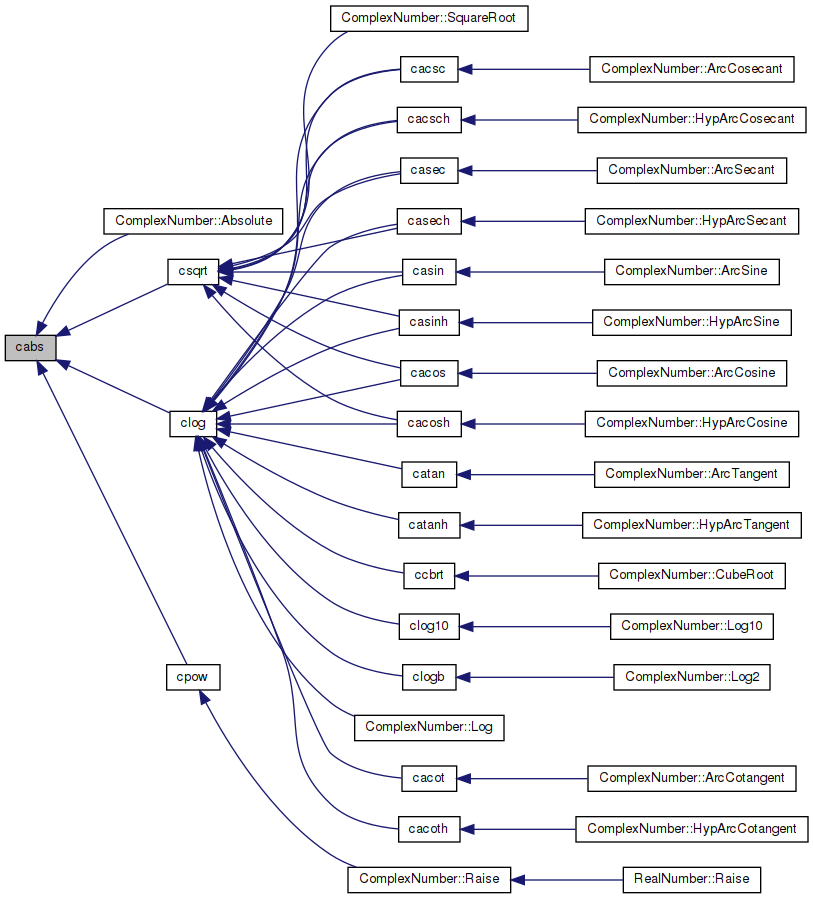## ◆ cacos()

 complex cacos ( complex z )

Inverse cosine of complex number.

Inverse cosine expressed using complex logarithms:

```arccos z = -i * log(z + i * sqrt(1 - z * z))
```

https://wikipedia.org/wiki/Inverse_trigonometric_functions#Logarithmic_forms

Definition at line 42 of file cacos.c.

References cadd(), clog(), cmul(), cpack(), csqrt(), and csub().

Referenced by ComplexNumber::ArcCosine().

43 {
44  complex a = cpack(1.0, 0.0);
45  complex i = cpack(0.0, 1.0);
46  complex j = cpack(0.0, -1.0);
47  complex p = csub(a, cmul(z, z));
48  complex q = clog(cadd(z, cmul(i, csqrt(p))));
49  complex w = cmul(j, q);
50  return w;
51 }
complex csub(complex y, complex z)
Subtraction of two complex numbers.
Definition: prim.c:130
Definition: mathi.h:48
complex cmul(complex y, complex z)
Multiplication of two complex numbers.
Definition: prim.c:140
complex cpack(double x, double y)
Pack two real numbers into a complex number.
Definition: prim.c:68
complex csqrt(complex z)
Square root of complex number.
Definition: csqrt.c:42
Definition: prim.c:120
complex clog(complex z)
Natural logarithm of a complex number.
Definition: clog.c:42
Here is the call graph for this function: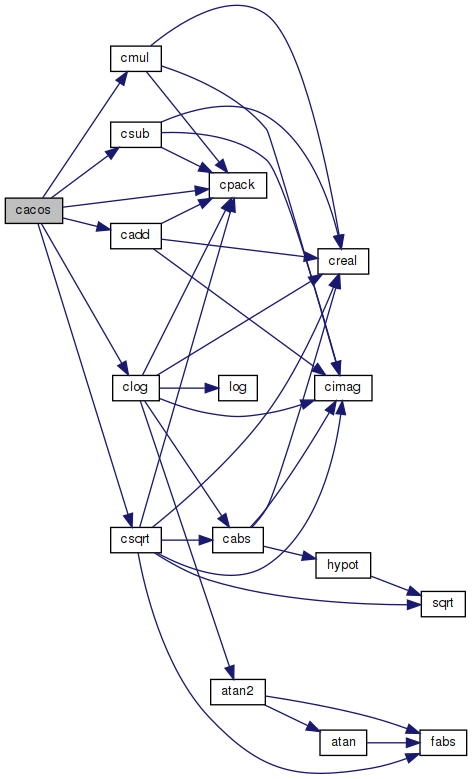Here is the caller graph for this function: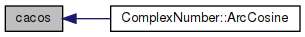## ◆ cacosh()

 complex cacosh ( complex z )

Inverse hyperbolic cosine of complex number.

Inverse hyperbolic cosine expressed using complex logarithms:

```acosh(z) = log(z + sqrt(z*z - 1))
```

https://wikipedia.org/wiki/Inverse_hyperbolic_function#Logarithmic_representation

Definition at line 42 of file cacosh.c.

References cadd(), clog(), cmul(), cpack(), csqrt(), and csub().

Referenced by ComplexNumber::HypArcCosine().

43 {
44  complex one = cpack(1.0, 0.0);
45  complex a = csub(cmul(z, z), one);
46  complex b = cadd(z, csqrt(a));
47  complex w = clog(b);
48  return w;
49 }
complex csub(complex y, complex z)
Subtraction of two complex numbers.
Definition: prim.c:130
Definition: mathi.h:48
complex cmul(complex y, complex z)
Multiplication of two complex numbers.
Definition: prim.c:140
complex cpack(double x, double y)
Pack two real numbers into a complex number.
Definition: prim.c:68
complex csqrt(complex z)
Square root of complex number.
Definition: csqrt.c:42
Definition: prim.c:120
#define one
Definition: ktan.c:67
complex clog(complex z)
Natural logarithm of a complex number.
Definition: clog.c:42
Here is the call graph for this function: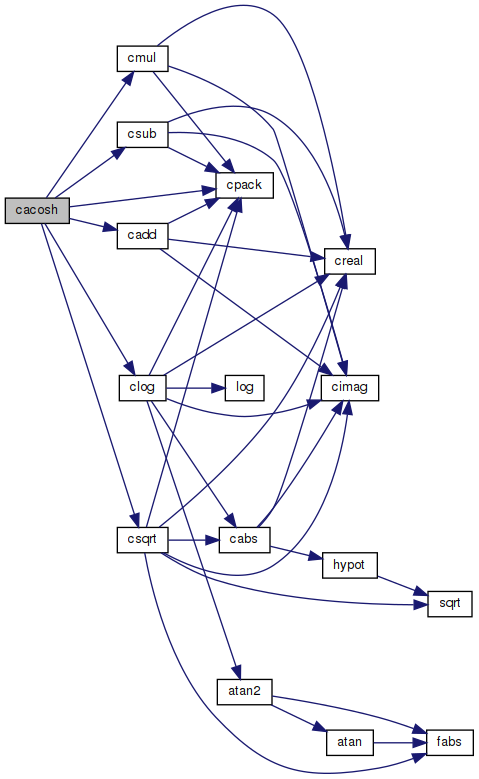Here is the caller graph for this function: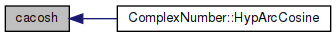## ◆ cacot()

 complex cacot ( complex z )

Inverse cotangent of complex number.

Inverse cotangent expressed using complex logarithms:

```arccot z = i/2 * (log(1 - i/z) - log(1 + i/z))
```

https://wikipedia.org/wiki/Inverse_trigonometric_functions#Logarithmic_forms

Definition at line 42 of file cacot.c.

References cadd(), cdiv(), clog(), cmul(), cpack(), and csub().

Referenced by ComplexNumber::ArcCotangent().

43 {
44  complex one = cpack(1.0, 0.0);
45  complex two = cpack(2.0, 0.0);
46  complex i = cpack(0.0, 1.0);
47  complex iz = cdiv(i, z);
48  complex p = clog(csub(one, iz));
49  complex q = clog(cadd(one, iz));
50  complex w = cmul(cdiv(i, two), csub(p, q));
51  return w;
52 }
complex csub(complex y, complex z)
Subtraction of two complex numbers.
Definition: prim.c:130
complex cdiv(complex y, complex z)
Division of two complex numbers.
Definition: prim.c:159
Definition: mathi.h:48
complex cmul(complex y, complex z)
Multiplication of two complex numbers.
Definition: prim.c:140
complex cpack(double x, double y)
Pack two real numbers into a complex number.
Definition: prim.c:68
Definition: prim.c:120
static const double two
Definition: pow.c:67
#define one
Definition: ktan.c:67
complex clog(complex z)
Natural logarithm of a complex number.
Definition: clog.c:42
Here is the call graph for this function: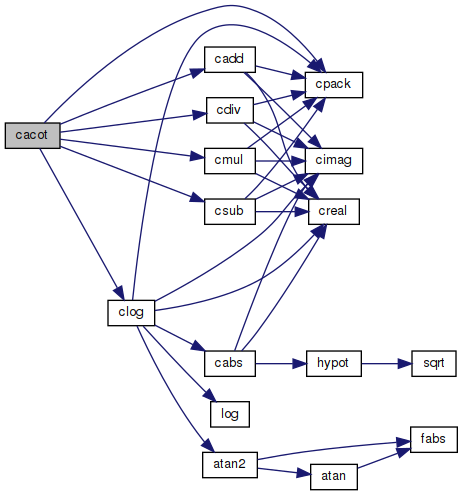Here is the caller graph for this function: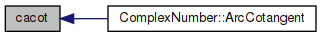## ◆ cacoth()

 complex cacoth ( complex z )

Inverse hyperbolic cotangent of complex number.

Inverse hyperbolic cotangent expressed using complex logarithms:

```acoth(z) = 1/2 * ((log(z + 1) - log(z - 1))
```

https://wikipedia.org/wiki/Inverse_hyperbolic_function#Logarithmic_representation

Definition at line 42 of file cacoth.c.

References cadd(), clog(), cmul(), cpack(), and csub().

Referenced by ComplexNumber::HypArcCotangent().

43 {
44  complex half = cpack(0.5, 0.0);
45  complex one = cpack(1.0, 0.0);
46  complex a = clog(cadd(z, one));
47  complex b = clog(csub(z, one));
48  complex c = csub(a, b);
49  complex w = cmul(half, c);
50  return w;
51 }
complex csub(complex y, complex z)
Subtraction of two complex numbers.
Definition: prim.c:130
Definition: mathi.h:48
complex cmul(complex y, complex z)
Multiplication of two complex numbers.
Definition: prim.c:140
complex cpack(double x, double y)
Pack two real numbers into a complex number.
Definition: prim.c:68
Definition: prim.c:120
#define one
Definition: ktan.c:67
complex clog(complex z)
Natural logarithm of a complex number.
Definition: clog.c:42
static const double half
Definition: cosh.c:54
Here is the call graph for this function: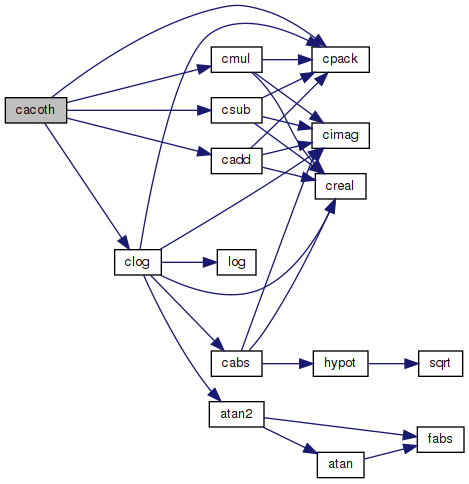Here is the caller graph for this function: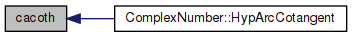## ◆ cacsc()

 complex cacsc ( complex z )

Inverse cosecant of complex number.

Inverse cosecant expressed using complex logarithms:

```arccsc z = -i * log(sqr(1 - 1/(z*z)) + i/z)
```

https://wikipedia.org/wiki/Inverse_trigonometric_functions#Logarithmic_forms

Definition at line 42 of file cacsc.c.

References cadd(), cdiv(), clog(), cmul(), cpack(), csqrt(), and csub().

Referenced by ComplexNumber::ArcCosecant().

43 {
44  complex one = cpack(1.0, 0.0);
45  complex i = cpack(0.0, 1.0);
46  complex j = cpack(0.0, -1.0);
47  complex iz = cdiv(i, z);
48  complex z2 = cmul(z, z);
49  complex p = cdiv(one, z2);
50  complex q = csqrt(csub(one, p));
51  complex w = cmul(j, clog(cadd(q, iz)));
52  return w;
53 }
complex csub(complex y, complex z)
Subtraction of two complex numbers.
Definition: prim.c:130
complex cdiv(complex y, complex z)
Division of two complex numbers.
Definition: prim.c:159
Definition: mathi.h:48
complex cmul(complex y, complex z)
Multiplication of two complex numbers.
Definition: prim.c:140
complex cpack(double x, double y)
Pack two real numbers into a complex number.
Definition: prim.c:68
complex csqrt(complex z)
Square root of complex number.
Definition: csqrt.c:42
Definition: prim.c:120
#define one
Definition: ktan.c:67
complex clog(complex z)
Natural logarithm of a complex number.
Definition: clog.c:42
Here is the call graph for this function: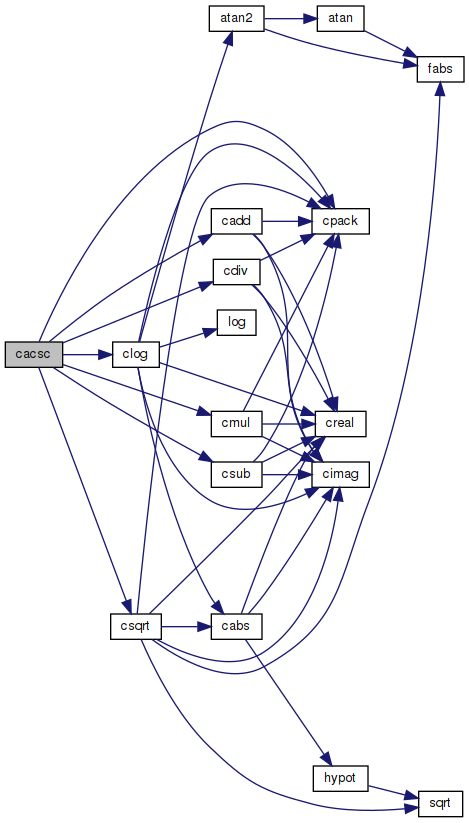Here is the caller graph for this function: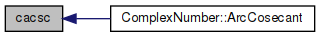## ◆ cacsch()

 complex cacsch ( complex z )

Inverse hyperbolic cosecant of complex number.

Inverse hyperbolic cosecant expressed using complex logarithms:

```acsch(z) = log(sqrt(1 + 1 / (z * z)) + 1/z)
```

https://wikipedia.org/wiki/Inverse_hyperbolic_function#Logarithmic_representation

Definition at line 42 of file cacsch.c.

References cadd(), clog(), cmul(), cpack(), creci(), and csqrt().

Referenced by ComplexNumber::HypArcCosecant().

43 {
44  complex one = cpack(1.0, 0.0);
45  complex a = creci(cmul(z, z));
46  complex b = csqrt(cadd(one, a));
47  complex c = cadd(b, creci(z));
48  complex w = clog(c);
49  return w;
50 }
Definition: mathi.h:48
complex cmul(complex y, complex z)
Multiplication of two complex numbers.
Definition: prim.c:140
complex cpack(double x, double y)
Pack two real numbers into a complex number.
Definition: prim.c:68
complex csqrt(complex z)
Square root of complex number.
Definition: csqrt.c:42
Definition: prim.c:120
#define one
Definition: ktan.c:67
complex creci(complex z)
Reciprocal value of complex number.
Definition: prim.c:181
complex clog(complex z)
Natural logarithm of a complex number.
Definition: clog.c:42
Here is the call graph for this function: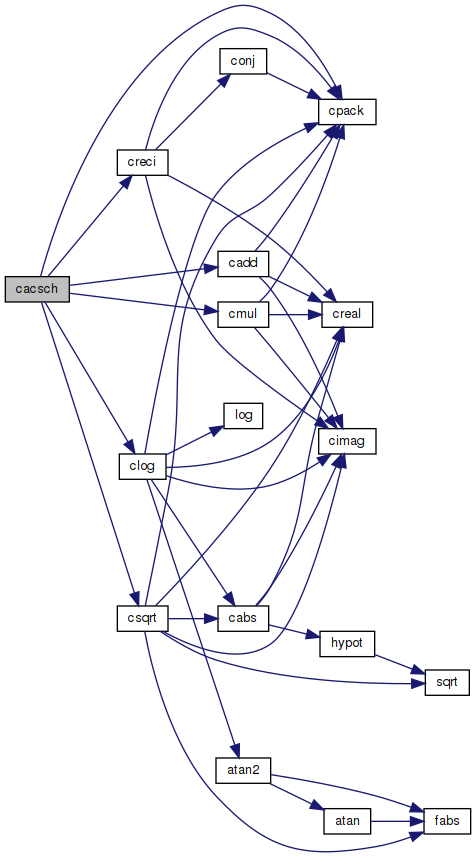Here is the caller graph for this function: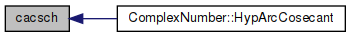complex cadd ( complex a, complex z )

Definition at line 120 of file prim.c.

References cimag(), cpack(), and creal().

Referenced by ComplexNumber::Add(), cacos(), cacosh(), cacot(), cacoth(), cacsc(), cacsch(), casec(), casech(), casin(), casinh(), catan(), and catanh().

121 {
122  complex w;
123  w = cpack(creal(y) + creal(z), cimag(y) + cimag(z));
124  return w;
125 }
Definition: mathi.h:48
complex cpack(double x, double y)
Pack two real numbers into a complex number.
Definition: prim.c:68
double cimag(complex z)
Imaginary part of complex number.
Definition: prim.c:46
double creal(complex z)
Real part of complex number.
Definition: prim.c:38
Here is the call graph for this function: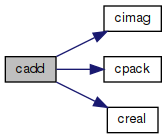Here is the caller graph for this function: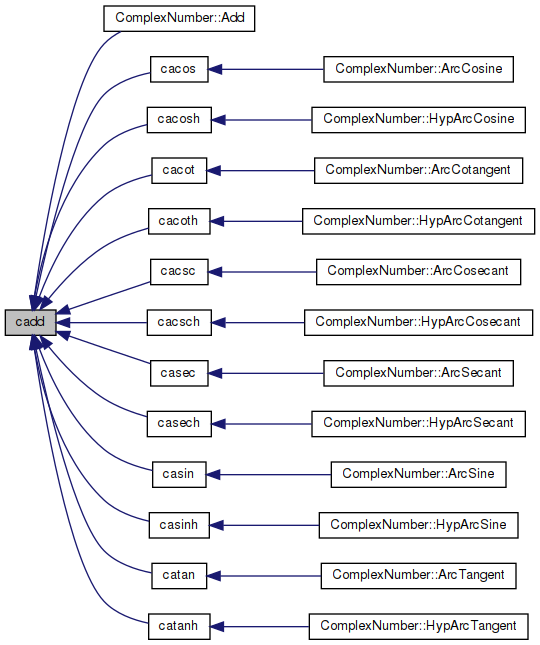## ◆ casec()

 complex casec ( complex z )

Inverse secant expressed using complex logarithms:

Inverse secant expressed using complex logarithms:

```arcsec z = -i * log(i * sqr(1 - 1/(z*z)) + 1/z)
```

https://wikipedia.org/wiki/Inverse_trigonometric_functions#Logarithmic_forms

Definition at line 42 of file casec.c.

References cadd(), cdiv(), clog(), cmul(), cpack(), creci(), csqrt(), and csub().

Referenced by ComplexNumber::ArcSecant().

43 {
44  complex one = cpack(1.0, 0.0);
45  complex i = cpack(0.0, 1.0);
46  complex j = cpack(0.0, -1.0);
47  complex rz = creci(z);
48  complex z2 = cmul(z, z);
49  complex p = cdiv(one, z2);
50  complex q = csqrt(csub(one, p));
51  complex w = cmul(j, clog(cadd(cmul(i, q), rz)));
52  return w;
53 }
complex csub(complex y, complex z)
Subtraction of two complex numbers.
Definition: prim.c:130
complex cdiv(complex y, complex z)
Division of two complex numbers.
Definition: prim.c:159
Definition: mathi.h:48
complex cmul(complex y, complex z)
Multiplication of two complex numbers.
Definition: prim.c:140
complex cpack(double x, double y)
Pack two real numbers into a complex number.
Definition: prim.c:68
complex csqrt(complex z)
Square root of complex number.
Definition: csqrt.c:42
Definition: prim.c:120
#define one
Definition: ktan.c:67
complex creci(complex z)
Reciprocal value of complex number.
Definition: prim.c:181
complex clog(complex z)
Natural logarithm of a complex number.
Definition: clog.c:42
Here is the call graph for this function: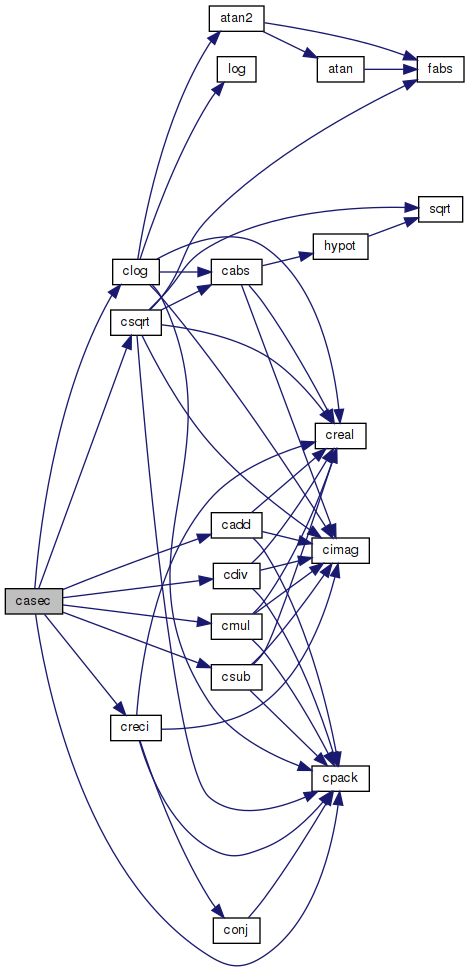Here is the caller graph for this function: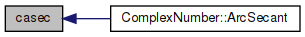## ◆ casech()

 complex casech ( complex z )

Inverse hyperbolic secant of complex numbers.

Inverse hyperbolic secant expressed using complex logarithms:

```asech(z) = log(sqrt(1 / (z * z) - 1) + 1/z)
```

https://wikipedia.org/wiki/Inverse_hyperbolic_function#Logarithmic_representation

Definition at line 42 of file casech.c.

References cadd(), clog(), cmul(), cpack(), creci(), csqrt(), and csub().

Referenced by ComplexNumber::HypArcSecant().

43 {
44  complex one = cpack(1.0, 0.0);
45  complex a = creci(cmul(z, z));
46  complex b = csqrt(csub(a, one));
47  complex c = cadd(b, creci(z));
48  complex w = clog(c);
49  return w;
50 }
complex csub(complex y, complex z)
Subtraction of two complex numbers.
Definition: prim.c:130
Definition: mathi.h:48
complex cmul(complex y, complex z)
Multiplication of two complex numbers.
Definition: prim.c:140
complex cpack(double x, double y)
Pack two real numbers into a complex number.
Definition: prim.c:68
complex csqrt(complex z)
Square root of complex number.
Definition: csqrt.c:42
Definition: prim.c:120
#define one
Definition: ktan.c:67
complex creci(complex z)
Reciprocal value of complex number.
Definition: prim.c:181
complex clog(complex z)
Natural logarithm of a complex number.
Definition: clog.c:42
Here is the call graph for this function: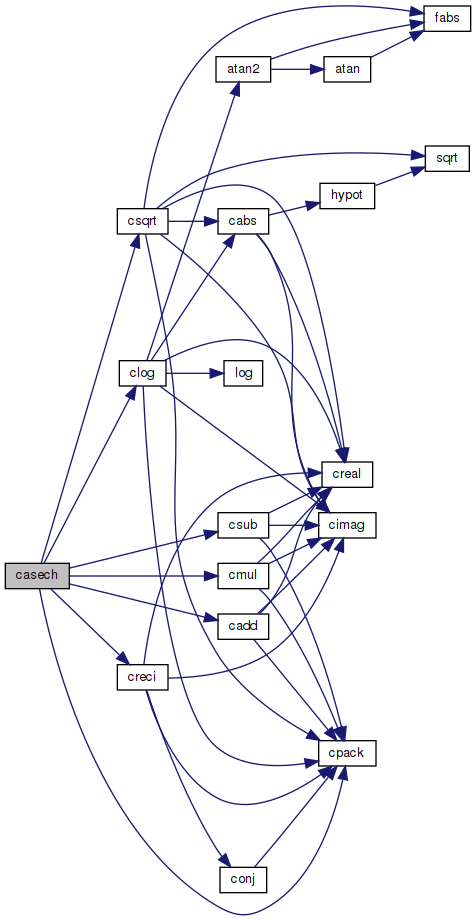Here is the caller graph for this function: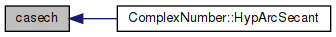## ◆ casin()

 complex casin ( complex z )

Inverse sine of complex number.

Inverse sine expressed using complex logarithms:

```arcsin z = -i * log(iz + sqrt(1 - z*z))
```

https://wikipedia.org/wiki/Inverse_trigonometric_functions#Logarithmic_forms

Definition at line 42 of file casin.c.

References cadd(), clog(), cmul(), cpack(), csqrt(), and csub().

Referenced by ComplexNumber::ArcSine().

43 {
44  complex one = cpack(1.0, 0.0);
45  complex i = cpack(0.0, 1.0);
46  complex j = cpack(0.0, -1.0);
47  complex iz = cmul(i, z);
48  complex z2 = cmul(z, z);
49  complex p = csqrt(csub(one, z2));
50  complex q = clog(cadd(iz, p));
51  complex w = cmul(j, q);
52  return w;
53 }
complex csub(complex y, complex z)
Subtraction of two complex numbers.
Definition: prim.c:130
Definition: mathi.h:48
complex cmul(complex y, complex z)
Multiplication of two complex numbers.
Definition: prim.c:140
complex cpack(double x, double y)
Pack two real numbers into a complex number.
Definition: prim.c:68
complex csqrt(complex z)
Square root of complex number.
Definition: csqrt.c:42
Definition: prim.c:120
#define one
Definition: ktan.c:67
complex clog(complex z)
Natural logarithm of a complex number.
Definition: clog.c:42
Here is the call graph for this function: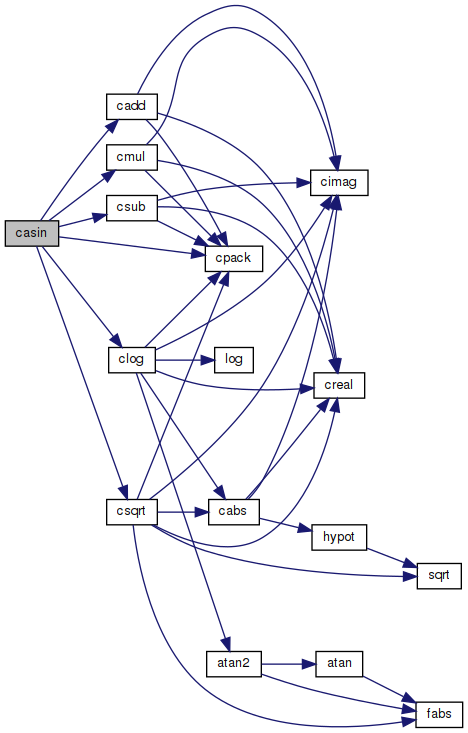Here is the caller graph for this function: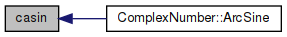## ◆ casinh()

 complex casinh ( complex z )

Inverse hyperbolic sine of complex number.

Inverse hyperbolic sine expressed using complex logarithms:

`asinh(z) = log(z + sqrt(z*z + 1))`
`With branch cuts: -i INF to -i and i to i INF`
```Domain: -INF to INF
Range:  -INF to INF
```

https://wikipedia.org/wiki/Inverse_hyperbolic_function#Logarithmic_representation

Definition at line 47 of file casinh.c.

References cadd(), clog(), cmul(), cpack(), and csqrt().

Referenced by ComplexNumber::HypArcSine().

48 {
49  // TODO: Fix branch cuts
50  complex one = cpack(1.0, 0.0);
51  complex a = cadd(cmul(z, z), one);
52  complex b = cadd(z, csqrt(a));
53  complex w = clog(b);
54  return w;
55 }
Definition: mathi.h:48
complex cmul(complex y, complex z)
Multiplication of two complex numbers.
Definition: prim.c:140
complex cpack(double x, double y)
Pack two real numbers into a complex number.
Definition: prim.c:68
complex csqrt(complex z)
Square root of complex number.
Definition: csqrt.c:42
Definition: prim.c:120
#define one
Definition: ktan.c:67
complex clog(complex z)
Natural logarithm of a complex number.
Definition: clog.c:42
Here is the call graph for this function: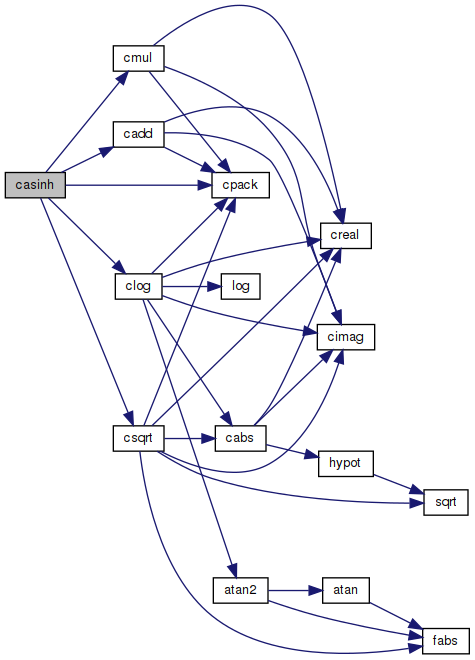Here is the caller graph for this function: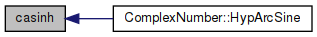## ◆ catan()

 complex catan ( complex z )

Inverse tangent of complex number.

Inverse tangent expressed using complex logarithms:

```atan(z) = i/2 * (log(1 - i * z) - log(1 + i * z))
```

https://wikipedia.org/wiki/Inverse_trigonometric_functions#Logarithmic_forms

Definition at line 42 of file catan.c.

References cadd(), cdiv(), clog(), cmul(), cpack(), and csub().

Referenced by ComplexNumber::ArcTangent().

43 {
44  complex one = cpack(1.0, 0.0);
45  complex two = cpack(2.0, 0.0);
46  complex i = cpack(0.0, 1.0);
47  complex iz = cmul(i, z);
48  complex p = clog(csub(one, iz));
49  complex q = clog(cadd(one, iz));
50  complex w = cmul(cdiv(i, two), csub(p, q));
51  return w;
52 }
complex csub(complex y, complex z)
Subtraction of two complex numbers.
Definition: prim.c:130
complex cdiv(complex y, complex z)
Division of two complex numbers.
Definition: prim.c:159
Definition: mathi.h:48
complex cmul(complex y, complex z)
Multiplication of two complex numbers.
Definition: prim.c:140
complex cpack(double x, double y)
Pack two real numbers into a complex number.
Definition: prim.c:68
Definition: prim.c:120
static const double two
Definition: pow.c:67
#define one
Definition: ktan.c:67
complex clog(complex z)
Natural logarithm of a complex number.
Definition: clog.c:42
Here is the call graph for this function: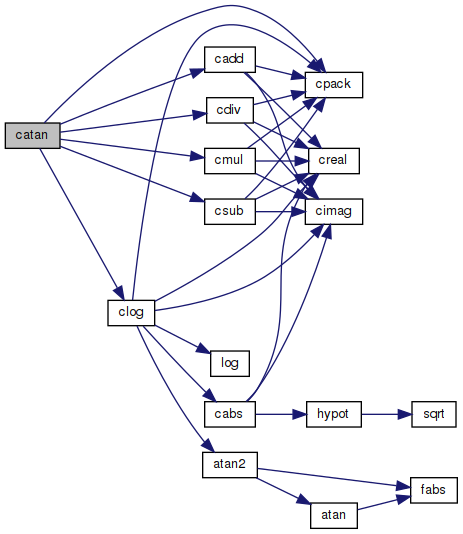Here is the caller graph for this function: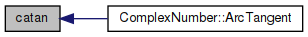## ◆ catanh()

 complex catanh ( complex z )

Inverse hyperbolic tangent of complex number.

Inverse hyperbolic tangent expressed using complex logarithms:

```atanh(z) = 1/2 * ((log(1 + z) - log(1 - z))
```

https://wikipedia.org/wiki/Inverse_hyperbolic_function#Logarithmic_representation

Definition at line 42 of file catanh.c.

References cadd(), clog(), cmul(), cpack(), and csub().

Referenced by ComplexNumber::HypArcTangent().

43 {
44  complex half = cpack(0.5, 0.0);
45  complex one = cpack(1.0, 0.0);
46  complex a = clog(cadd(one, z));
47  complex b = clog(csub(one, z));
48  complex c = csub(a, b);
49  complex w = cmul(half, c);
50  return w;
51 }
complex csub(complex y, complex z)
Subtraction of two complex numbers.
Definition: prim.c:130
Definition: mathi.h:48
complex cmul(complex y, complex z)
Multiplication of two complex numbers.
Definition: prim.c:140
complex cpack(double x, double y)
Pack two real numbers into a complex number.
Definition: prim.c:68
Definition: prim.c:120
#define one
Definition: ktan.c:67
complex clog(complex z)
Natural logarithm of a complex number.
Definition: clog.c:42
static const double half
Definition: cosh.c:54
Here is the call graph for this function: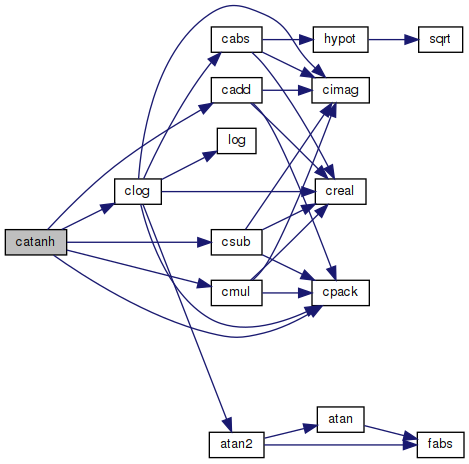Here is the caller graph for this function: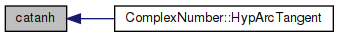## ◆ ccbrt()

 complex ccbrt ( complex z )

Cube root of complex number.

```cbrt z = exp(1/3 * log(z))
```

https://wikipedia.org/wiki/Cube_root

Definition at line 41 of file ccbrt.c.

References cexp(), clog(), cmul(), and cpack().

Referenced by ComplexNumber::CubeRoot().

42 {
43  complex onethird = cpack(1.0 / 3.0, 0.0);
44  complex a = cmul(onethird, clog(z));
45  complex w = cexp(a);
46  return w;
47 }
Definition: mathi.h:48
complex cmul(complex y, complex z)
Multiplication of two complex numbers.
Definition: prim.c:140
complex cpack(double x, double y)
Pack two real numbers into a complex number.
Definition: prim.c:68
complex cexp(complex z)
Returns e to the power of a complex number.
Definition: cexp.c:42
complex clog(complex z)
Natural logarithm of a complex number.
Definition: clog.c:42
Here is the call graph for this function: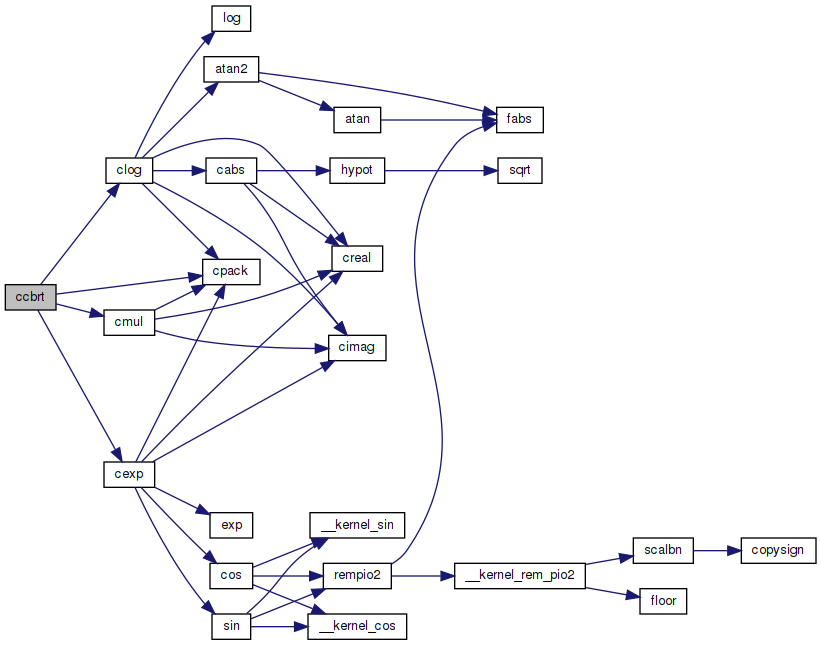Here is the caller graph for this function: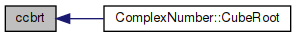## ◆ cceil()

 complex cceil ( complex z )

Ceiling value of complex number.

Definition at line 100 of file prim.c.

References ceil(), cimag(), cpack(), and creal().

Referenced by ComplexNumber::Ceiling().

101 {
102  complex w;
103  w = cpack(ceil(creal(z)), ceil(cimag(z)));
104  return w;
105 }
Definition: mathi.h:48
complex cpack(double x, double y)
Pack two real numbers into a complex number.
Definition: prim.c:68
double ceil(double x)
Ceiling function.
Definition: ceil.c:63
double cimag(complex z)
Imaginary part of complex number.
Definition: prim.c:46
double creal(complex z)
Real part of complex number.
Definition: prim.c:38
Here is the call graph for this function: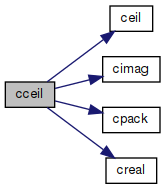Here is the caller graph for this function: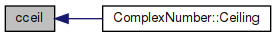## ◆ ccos()

 complex ccos ( complex z )

Cosine of complex number.

```a+bi
real =  cos(a) * cosh(b)
imag = -sin(a) * sinh(b)
```

Definition at line 45 of file ccos.c.

References cchsh(), cimag(), cos(), cpack(), creal(), and sin().

Referenced by ComplexNumber::Cosine().

46 {
47  complex w;
48  double a, b;
49  double ch, sh;
50
51  a = creal(z);
52  b = cimag(z);
53  cchsh(b, &ch, &sh);
54  w = cpack((cos(a) * ch), (-sin(a) * sh));
55
56  return w;
57 }
double sin(double x)
Sine function.
Definition: sin.c:86
Definition: mathi.h:48
complex cpack(double x, double y)
Pack two real numbers into a complex number.
Definition: prim.c:68
double cimag(complex z)
Imaginary part of complex number.
Definition: prim.c:46
double cos(double x)
Cosine function.
Definition: cos.c:87
void cchsh(double x, double *c, double *s)
Calculate cosh and sinh.
Definition: prim.c:197
double creal(complex z)
Real part of complex number.
Definition: prim.c:38
Here is the call graph for this function: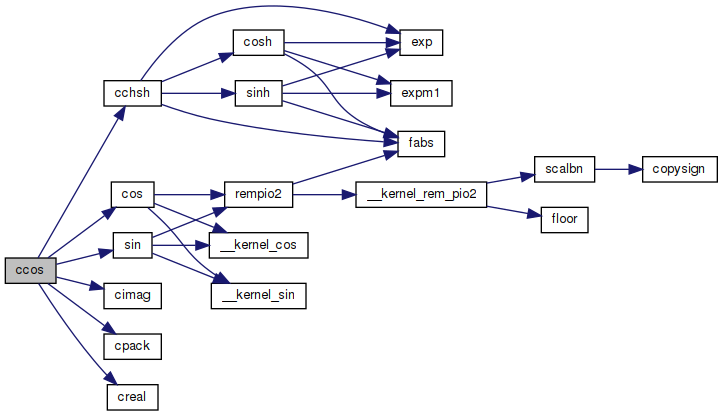Here is the caller graph for this function: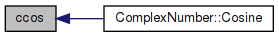## ◆ ccosh()

 complex ccosh ( complex z )

Hyperbolic cosine of a complex number.

```a+bi
real = cosh(a) * cos(b)
imag = sinh(a) * sin(b)
```

Definition at line 48 of file ccosh.c.

References cchsh(), cimag(), cos(), cpack(), creal(), and sin().

Referenced by ComplexNumber::HypCosine().

49 {
50  complex w;
51  double a, b;
52  double ch, sh;
53
54  a = creal(z);
55  b = cimag(z);
56  cchsh(a, &ch, &sh);
57  w = cpack(cos(b) * ch, sin(b) * sh);
58
59  return w;
60 }
double sin(double x)
Sine function.
Definition: sin.c:86
Definition: mathi.h:48
complex cpack(double x, double y)
Pack two real numbers into a complex number.
Definition: prim.c:68
double cimag(complex z)
Imaginary part of complex number.
Definition: prim.c:46
double cos(double x)
Cosine function.
Definition: cos.c:87
void cchsh(double x, double *c, double *s)
Calculate cosh and sinh.
Definition: prim.c:197
double creal(complex z)
Real part of complex number.
Definition: prim.c:38
Here is the call graph for this function: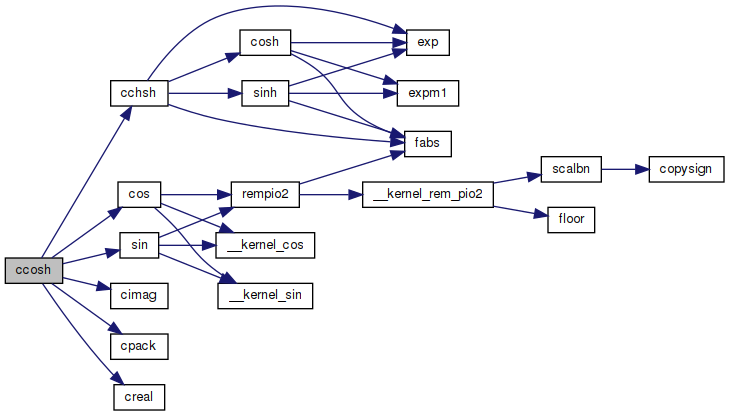Here is the caller graph for this function: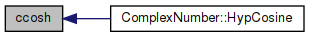## ◆ ccot()

 complex ccot ( complex z )

Cotangent of a complex number.

Calculated as in Open Office:

```a+bi
sin(2.0 * a)
real  = ------------------------------
cosh(2.0 * b) - cos(2.0 * a)```
```               -sinh(2.0 * b)
imag  = ------------------------------
cosh(2.0 * b) - cos(2.0 * a)
```

Definition at line 48 of file ccot.c.

References cimag(), cos(), cosh(), cpack(), creal(), sin(), and sinh().

Referenced by ComplexNumber::Cotangent().

49 {
50  complex w;
51  double a, b;
52  double d;
53
54  a = creal(z);
55  b = cimag(z);
56  d = cosh(2.0 * b) - cos(2.0 * a);
57
58  if (d == 0.0)
59  {
60  w = cpack((double)INFP, (double)INFP);
61  }
62  else
63  {
64  w = cpack((sin(2.0 * a) / d), (-sinh(2.0 * b) / d));
65  }
66
67  return w;
68 }
double sin(double x)
Sine function.
Definition: sin.c:86
double cosh(double x)
Hyperbolic cosine function.
Definition: cosh.c:83
Definition: mathi.h:48
complex cpack(double x, double y)
Pack two real numbers into a complex number.
Definition: prim.c:68
double cimag(complex z)
Imaginary part of complex number.
Definition: prim.c:46
double cos(double x)
Cosine function.
Definition: cos.c:87
#define INFP
Definition: mathr.h:51
double sinh(double x)
Hyperbolic sine function.
Definition: sinh.c:77
double creal(complex z)
Real part of complex number.
Definition: prim.c:38
Here is the call graph for this function: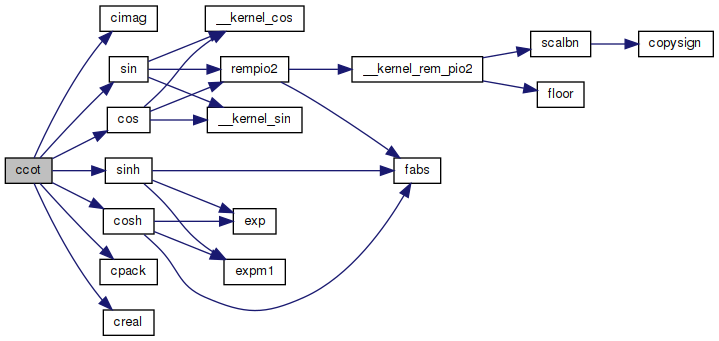Here is the caller graph for this function: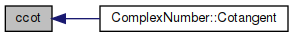## ◆ ccoth()

 complex ccoth ( complex z )

Hyperbolic cotangent of a complex number.

```acoth(z) = 0.5 * (log(1 + 1/z) - log(1 - 1/z))
```

or

```a+bi
sinh(2.0 * a)
real  = ---------------------------—
cosh(2.0 * a) - cos(2.0 * b)
-sin(2.0 * b)

imag  = ---------------------------—
cosh(2.0 * a) - cos(2.0 * b)
```

Definition at line 50 of file ccoth.c.

References cimag(), cos(), cosh(), cpack(), creal(), sin(), and sinh().

Referenced by ComplexNumber::HypCotangent().

51 {
52  complex w;
53  double a, b;
54  double d;
55
56  a = creal(z);
57  b = cimag(z);
58  d = cosh(2.0 * a) - cos(2.0 * b);
59  w = cpack(sinh(2.0 * a) / d, -sin(2.0 * b) / d);
60
61  return w;
62 }
double sin(double x)
Sine function.
Definition: sin.c:86
double cosh(double x)
Hyperbolic cosine function.
Definition: cosh.c:83
Definition: mathi.h:48
complex cpack(double x, double y)
Pack two real numbers into a complex number.
Definition: prim.c:68
double cimag(complex z)
Imaginary part of complex number.
Definition: prim.c:46
double cos(double x)
Cosine function.
Definition: cos.c:87
double sinh(double x)
Hyperbolic sine function.
Definition: sinh.c:77
double creal(complex z)
Real part of complex number.
Definition: prim.c:38
Here is the call graph for this function: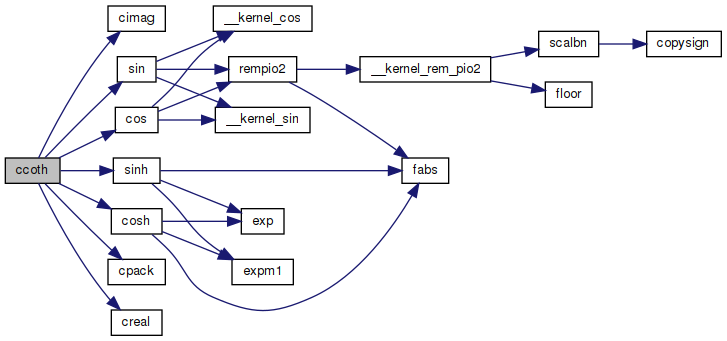Here is the caller graph for this function: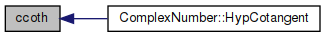## ◆ ccsc()

 complex ccsc ( complex z )

Cosecant of a complex number.

Calculated as in Open Office:

```a+bi
2.0 * sin(a) * cosh(b)
real  = ------------------------------
cosh(2.0 * b) - cos(2.0 * a)```
```           -2.0 * cos(a) * sinh(b)
imag  = ------------------------------
cosh(2.0 * b) - cos(2.0 * a)
```

Definition at line 48 of file ccsc.c.

References cimag(), cos(), cosh(), cpack(), creal(), sin(), and sinh().

Referenced by ComplexNumber::Cosecant().

49 {
50  complex w;
51  double a, b;
52  double d;
53
54  a = creal(z);
55  b = cimag(z);
56  d = cosh(2.0 * b) - cos(2.0 * a);
57
58  if (d == 0.0)
59  {
60  w = cpack((double)INFP, (double)INFP);
61  }
62  else
63  {
64  w = cpack((2.0 * sin(a) * cosh(b) / d), (-2.0 * cos(a) * sinh(b) / d));
65  }
66
67  return w;
68 }
double sin(double x)
Sine function.
Definition: sin.c:86
double cosh(double x)
Hyperbolic cosine function.
Definition: cosh.c:83
Definition: mathi.h:48
complex cpack(double x, double y)
Pack two real numbers into a complex number.
Definition: prim.c:68
double cimag(complex z)
Imaginary part of complex number.
Definition: prim.c:46
double cos(double x)
Cosine function.
Definition: cos.c:87
#define INFP
Definition: mathr.h:51
double sinh(double x)
Hyperbolic sine function.
Definition: sinh.c:77
double creal(complex z)
Real part of complex number.
Definition: prim.c:38
Here is the call graph for this function: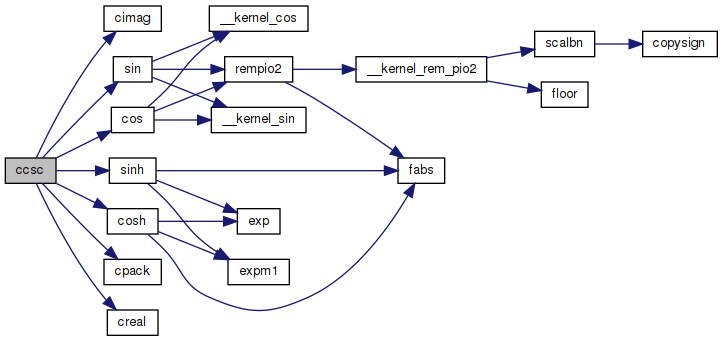Here is the caller graph for this function: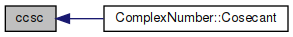## ◆ ccsch()

 complex ccsch ( complex z )

Hyperbolic secant of a complex number.

Calculated as in Open Office:

```a+bi
2.0 * sinh(a) * cos(b)
real  = ------------------------------
cosh(2.0 * a) - cos(2.0 * b)```
```        -2.0 * cosh(2.0 * a) * sin(b)
imag  = ------------------------------
cosh(2.0 * a) - cos(2.0 * b)
```

Definition at line 48 of file ccsch.c.

References cimag(), cos(), cosh(), cpack(), creal(), sin(), and sinh().

Referenced by ComplexNumber::HypCosecant().

49 {
50  complex w;
51  double a, b;
52  double d;
53
54  a = creal(z);
55  b = cimag(z);
56  d = cosh(2.0 * a) - cos(2.0 * b);
57  w = cpack((2.0 * sinh(a) * cos(b) / d), (-2.0 * cosh(a) * sin(b) / d));
58
59  return w;
60 }
double sin(double x)
Sine function.
Definition: sin.c:86
double cosh(double x)
Hyperbolic cosine function.
Definition: cosh.c:83
Definition: mathi.h:48
complex cpack(double x, double y)
Pack two real numbers into a complex number.
Definition: prim.c:68
double cimag(complex z)
Imaginary part of complex number.
Definition: prim.c:46
double cos(double x)
Cosine function.
Definition: cos.c:87
double sinh(double x)
Hyperbolic sine function.
Definition: sinh.c:77
double creal(complex z)
Real part of complex number.
Definition: prim.c:38
Here is the call graph for this function: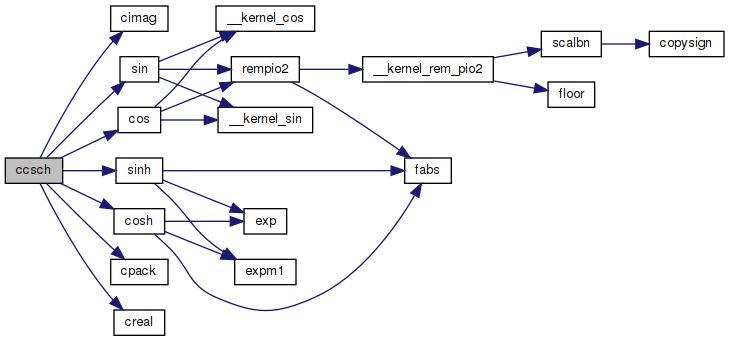Here is the caller graph for this function: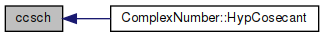## ◆ cdiv()

 complex cdiv ( complex a, complex z )

Division of two complex numbers.

Definition at line 159 of file prim.c.

References cimag(), cpack(), and creal().

Referenced by cacot(), cacsc(), casec(), catan(), clog10(), clogb(), and ComplexNumber::Div().

160 {
161  complex w;
162  double a, b, c, d;
163  double q, v, x;
164
165  a = creal(y);
166  b = cimag(y);
167  c = creal(z);
168  d = cimag(z);
169
170  q = c * c + d * d;
171  v = a * c + b * d;
172  x = b * c - a * d;
173
174  w = cpack(v / q, x / q);
175  return w;
176 }
Definition: mathi.h:48
complex cpack(double x, double y)
Pack two real numbers into a complex number.
Definition: prim.c:68
double cimag(complex z)
Imaginary part of complex number.
Definition: prim.c:46
double creal(complex z)
Real part of complex number.
Definition: prim.c:38
Here is the call graph for this function: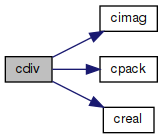Here is the caller graph for this function: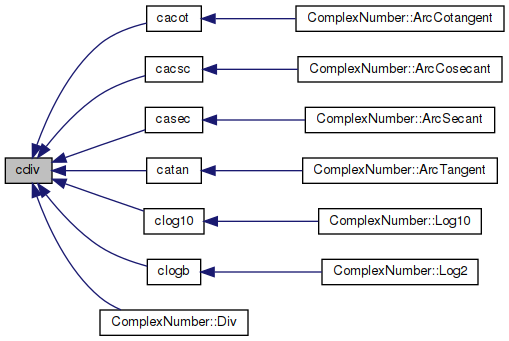## ◆ cexp()

 complex cexp ( complex z )

Returns e to the power of a complex number.

Definition at line 42 of file cexp.c.

References cimag(), cos(), cpack(), creal(), exp(), and sin().

Referenced by ccbrt().

43 {
44  complex w;
45  double r, x, y;
46  x = creal(z);
47  y = cimag(z);
48  r = exp(x);
49  w = cpack(r * cos(y), r * sin(y));
50  return w;
51 }
double sin(double x)
Sine function.
Definition: sin.c:86
Definition: mathi.h:48
complex cpack(double x, double y)
Pack two real numbers into a complex number.
Definition: prim.c:68
double cimag(complex z)
Imaginary part of complex number.
Definition: prim.c:46
double cos(double x)
Cosine function.
Definition: cos.c:87
double creal(complex z)
Real part of complex number.
Definition: prim.c:38
double exp(double x)
Returns the exponential of x.
Definition: exp.c:138
Here is the call graph for this function: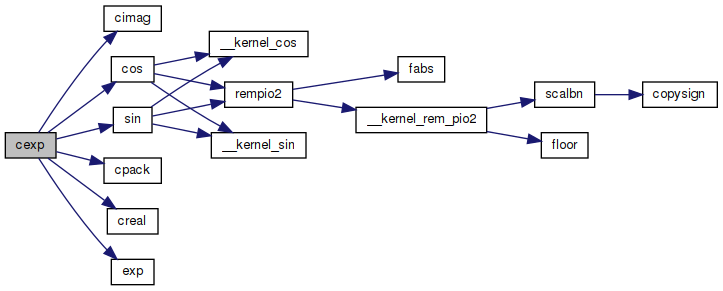Here is the caller graph for this function: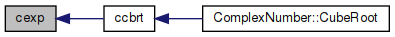## ◆ cfloor()

 complex cfloor ( complex z )

Floor value of complex number.

Definition at line 90 of file prim.c.

References cimag(), cpack(), creal(), and floor().

Referenced by ComplexNumber::Floor().

91 {
92  complex w;
93  w = cpack(floor(creal(z)), floor(cimag(z)));
94  return w;
95 }
Definition: mathi.h:48
complex cpack(double x, double y)
Pack two real numbers into a complex number.
Definition: prim.c:68
double cimag(complex z)
Imaginary part of complex number.
Definition: prim.c:46
double creal(complex z)
Real part of complex number.
Definition: prim.c:38
double floor(double x)
Floor function.
Definition: floor.c:62
Here is the call graph for this function: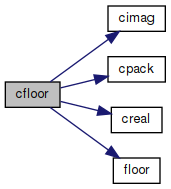Here is the caller graph for this function: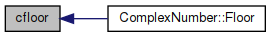## ◆ cimag()

 double cimag ( complex z )

Imaginary part of complex number.

Definition at line 46 of file prim.c.

47 {
48  return (IMAG_PART(z));
49 }
#define IMAG_PART(z)
Definition: prim.c:33
Here is the caller graph for this function: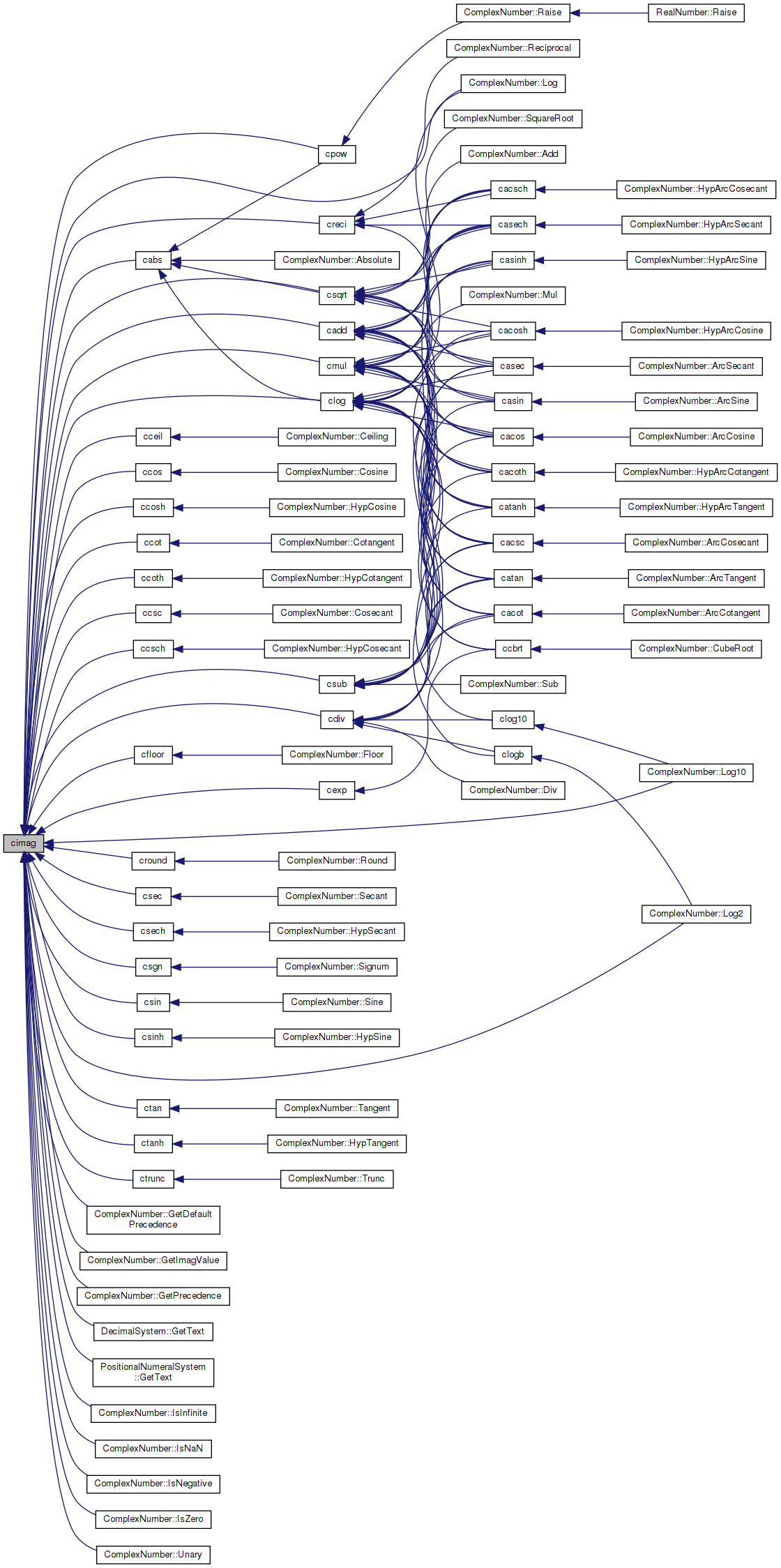## ◆ clog()

 complex clog ( complex z )

Natural logarithm of a complex number.

Definition at line 42 of file clog.c.

References atan2(), cabs(), cimag(), cpack(), creal(), and log().

Referenced by cacos(), cacosh(), cacot(), cacoth(), cacsc(), cacsch(), casec(), casech(), casin(), casinh(), catan(), catanh(), ccbrt(), clog10(), clogb(), and ComplexNumber::Log().

43 {
44  complex w;
45  double p, q;
46  p = log(cabs(z));
47  q = atan2(cimag(z), creal(z));
48  w = cpack(p, q);
49  return w;
50 }
Definition: mathi.h:48
complex cpack(double x, double y)
Pack two real numbers into a complex number.
Definition: prim.c:68
double cimag(complex z)
Imaginary part of complex number.
Definition: prim.c:46
double atan2(double y, double x)
Inverse tangent function.
Definition: atan2.c:87
double creal(complex z)
Real part of complex number.
Definition: prim.c:38
double log(double x)
Natural logarithm function (base e)
Definition: log.c:109
double cabs(complex z)
Absolute value of complex number.
Definition: prim.c:54
Here is the call graph for this function: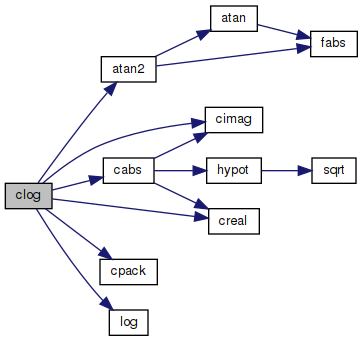Here is the caller graph for this function: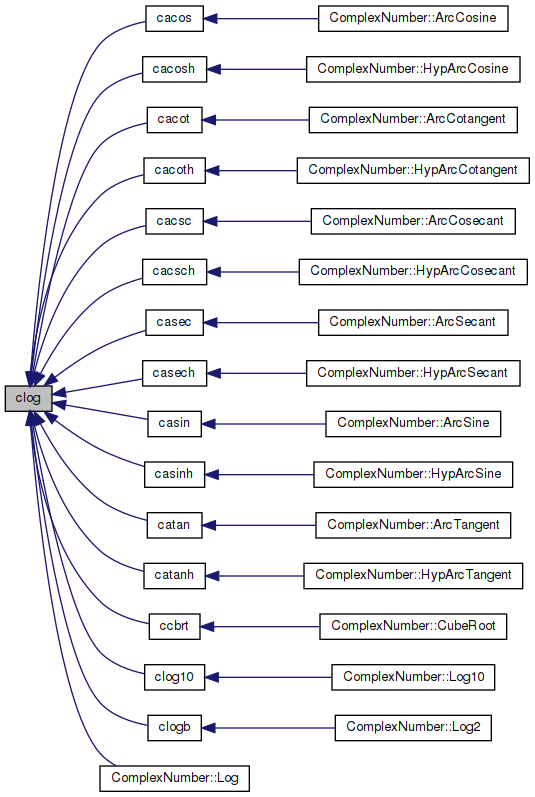## ◆ clog10()

 complex clog10 ( complex z )

Base 10 logarithmic value of complex number.

```log z = log(z) / log(10)
```

https://wikipedia.org/wiki/Complex_logarithm

Definition at line 41 of file clog10.c.

References cdiv(), clog(), and cpack().

Referenced by ComplexNumber::Log10().

42 {
43  complex teen = cpack(10.0, 0.0);
44  complex w = cdiv(clog(z), clog(teen));
45  return w;
46 }
complex cdiv(complex y, complex z)
Division of two complex numbers.
Definition: prim.c:159
Definition: mathi.h:48
complex cpack(double x, double y)
Pack two real numbers into a complex number.
Definition: prim.c:68
complex clog(complex z)
Natural logarithm of a complex number.
Definition: clog.c:42
Here is the call graph for this function: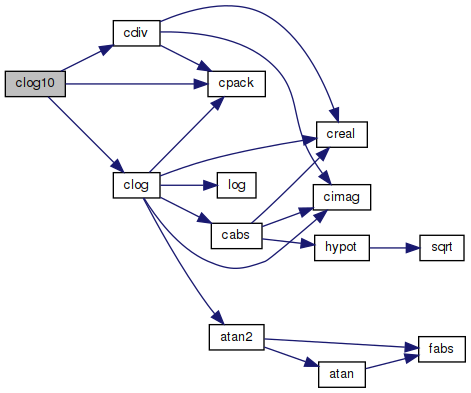Here is the caller graph for this function: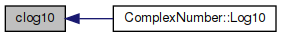## ◆ clogb()

 complex clogb ( complex z )

Base 2 logarithmic value of complex number.

```lb z = log(z) / log(2)
```

https://wikipedia.org/wiki/Complex_logarithm

Definition at line 41 of file clogb.c.

References cdiv(), clog(), and cpack().

Referenced by ComplexNumber::Log2().

42 {
43  complex two = cpack(2.0, 0.0);
44  complex w = cdiv(clog(z), clog(two));
45  return w;
46 }
complex cdiv(complex y, complex z)
Division of two complex numbers.
Definition: prim.c:159
Definition: mathi.h:48
complex cpack(double x, double y)
Pack two real numbers into a complex number.
Definition: prim.c:68
static const double two
Definition: pow.c:67
complex clog(complex z)
Natural logarithm of a complex number.
Definition: clog.c:42
Here is the call graph for this function: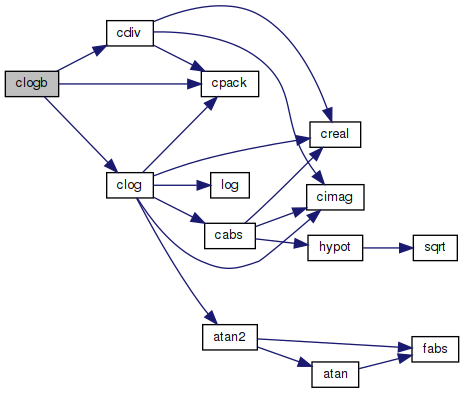Here is the caller graph for this function: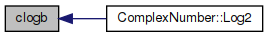## ◆ cmul()

 complex cmul ( complex a, complex z )

Multiplication of two complex numbers.

Definition at line 140 of file prim.c.

References cimag(), cpack(), and creal().

Referenced by cacos(), cacosh(), cacot(), cacoth(), cacsc(), cacsch(), casec(), casech(), casin(), casinh(), catan(), catanh(), ccbrt(), and ComplexNumber::Mul().

141 {
142  complex w;
143  double a, b, c, d;
144
145  // (a+bi)(c+di)
146  a = creal(y);
147  b = cimag(y);
148  c = creal(z);
149  d = cimag(z);
150
151  // (ac -bd) + (ad + bc)i
152  w = cpack(a * c - b * d, a * d + b * c);
153  return w;
154 }
Definition: mathi.h:48
complex cpack(double x, double y)
Pack two real numbers into a complex number.
Definition: prim.c:68
double cimag(complex z)
Imaginary part of complex number.
Definition: prim.c:46
double creal(complex z)
Real part of complex number.
Definition: prim.c:38
Here is the call graph for this function: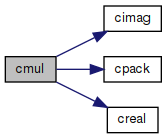Here is the caller graph for this function: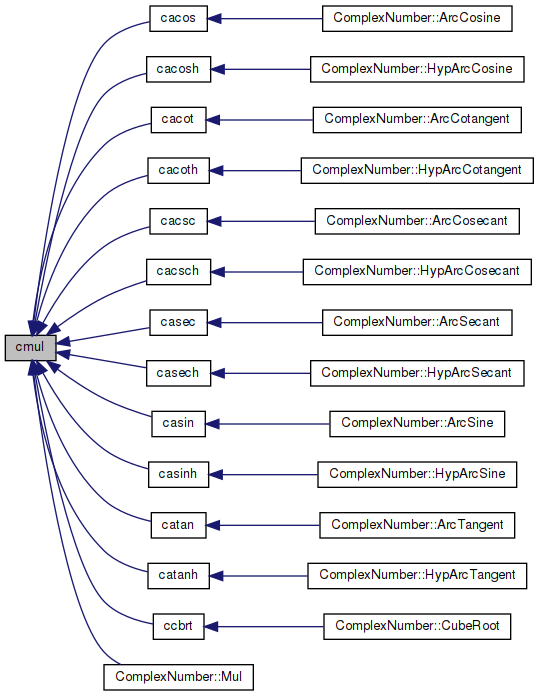## ◆ conj()

 complex conj ( complex z )

Definition at line 59 of file prim.c.

References cpack().

Referenced by creci().

60 {
61  IMAG_PART(z) = -IMAG_PART(z);
62  return cpack(REAL_PART(z), IMAG_PART(z));
63 }
complex cpack(double x, double y)
Pack two real numbers into a complex number.
Definition: prim.c:68
#define REAL_PART(z)
Definition: prim.c:32
#define IMAG_PART(z)
Definition: prim.c:33
Here is the call graph for this function: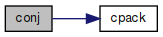Here is the caller graph for this function: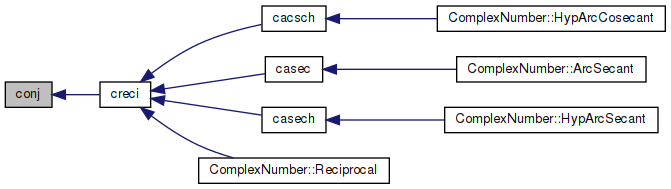## ◆ cpack()

 complex cpack ( double x, double y )

Pack two real numbers into a complex number.

Definition at line 68 of file prim.c.

69 {
70  complex z;
71
72  REAL_PART(z) = x;
73  IMAG_PART(z) = y;
74  return (z);
75 }
Definition: mathi.h:48
#define REAL_PART(z)
Definition: prim.c:32
#define IMAG_PART(z)
Definition: prim.c:33
Here is the caller graph for this function: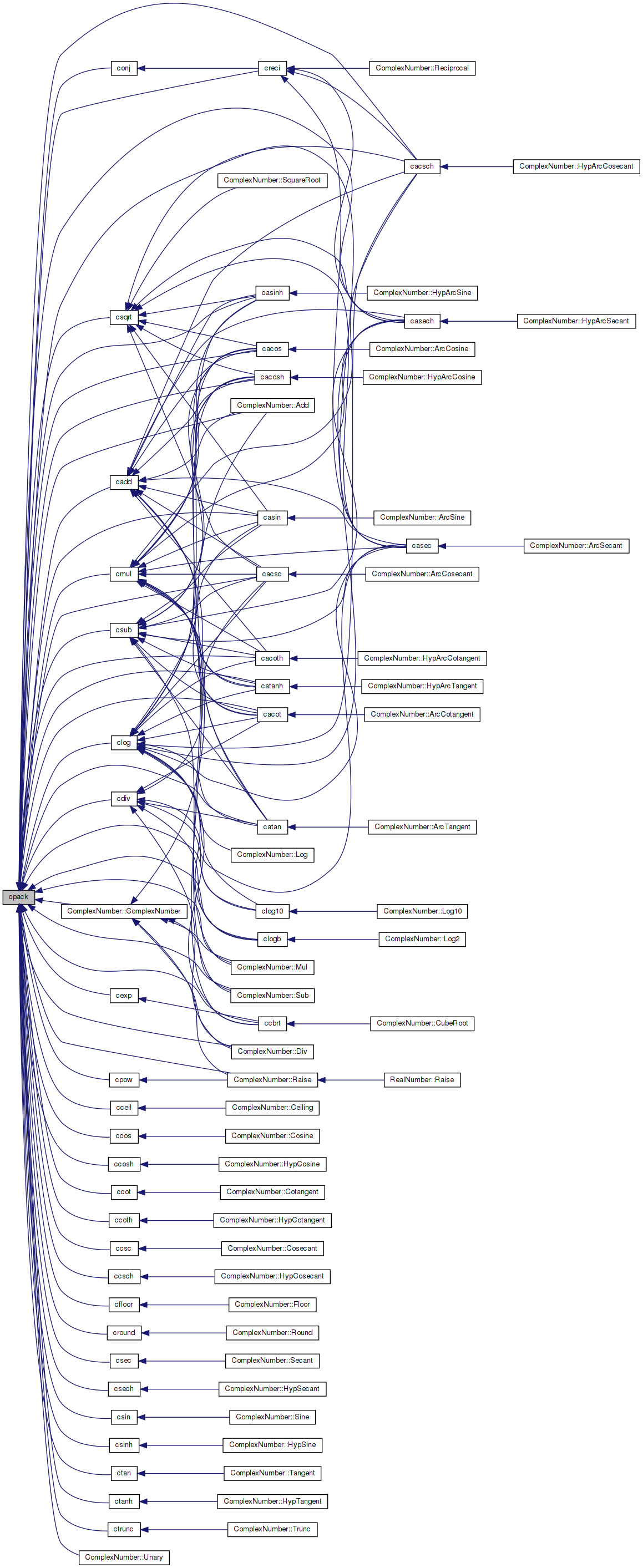## ◆ cpow()

 complex cpow ( complex x, complex z )

Complex number raised to a power.

Definition at line 42 of file cpow.c.

References atan2(), cabs(), cimag(), cos(), cpack(), creal(), exp(), log(), pow(), and sin().

Referenced by ComplexNumber::Raise().

43 {
44  complex w;
45  double x, y, r, theta, absa, arga;
46
47  x = creal(z);
48  y = cimag(z);
49  absa = cabs(a);
50  if (absa == 0.0)
51  {
52  return cpack(0.0, + 0.0);
53  }
54  arga = atan2(cimag(a), creal(a));
55
56  r = pow(absa, x);
57  theta = x * arga;
58  if (y != 0.0)
59  {
60  r = r * exp(-y * arga);
61  theta = theta + y * log(absa);
62  }
63
64  w = cpack(r * cos(theta), r * sin(theta));
65  return w;
66 }
double sin(double x)
Sine function.
Definition: sin.c:86
Definition: mathi.h:48
complex cpack(double x, double y)
Pack two real numbers into a complex number.
Definition: prim.c:68
double cimag(complex z)
Imaginary part of complex number.
Definition: prim.c:46
double cos(double x)
Cosine function.
Definition: cos.c:87
double atan2(double y, double x)
Inverse tangent function.
Definition: atan2.c:87
double creal(complex z)
Real part of complex number.
Definition: prim.c:38
double pow(double x, double y)
Expontation function.
Definition: pow.c:138
double exp(double x)
Returns the exponential of x.
Definition: exp.c:138
double log(double x)
Natural logarithm function (base e)
Definition: log.c:109
double cabs(complex z)
Absolute value of complex number.
Definition: prim.c:54
Here is the call graph for this function: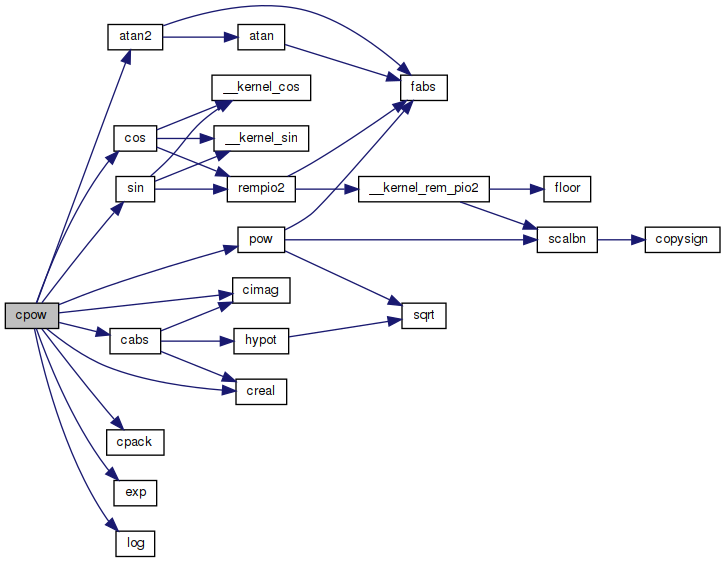Here is the caller graph for this function: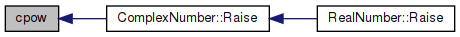## ◆ creal()

 double creal ( complex z )

Real part of complex number.

Definition at line 38 of file prim.c.

39 {
40  return (REAL_PART(z));
41 }
#define REAL_PART(z)
Definition: prim.c:32
Here is the caller graph for this function: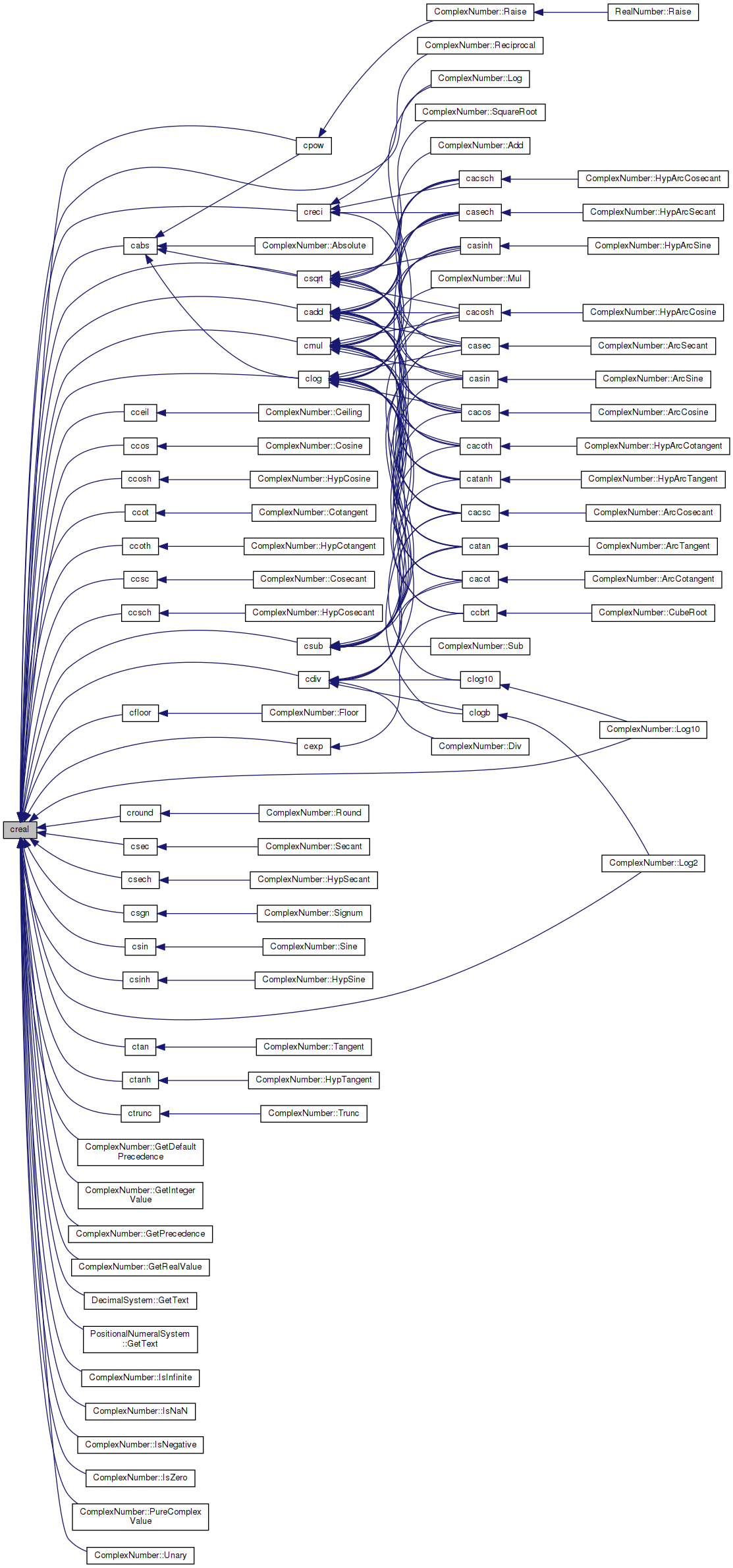## ◆ creci()

 complex creci ( complex z )

Reciprocal value of complex number.

Definition at line 181 of file prim.c.

References cimag(), conj(), cpack(), and creal().

Referenced by cacsch(), casec(), casech(), and ComplexNumber::Reciprocal().

182 {
183  complex w;
184  double q, a, b;
185
186  a = creal(z);
187  b = cimag(conj(z));
188  q = a * a + b * b;
189  w = cpack(a / q, b / q);
190
191  return w;
192 }
Definition: mathi.h:48
complex cpack(double x, double y)
Pack two real numbers into a complex number.
Definition: prim.c:68
double cimag(complex z)
Imaginary part of complex number.
Definition: prim.c:46
double creal(complex z)
Real part of complex number.
Definition: prim.c:38
complex conj(complex z)
Definition: prim.c:59
Here is the call graph for this function: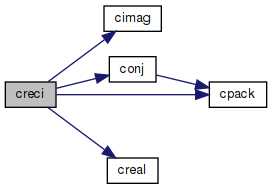Here is the caller graph for this function: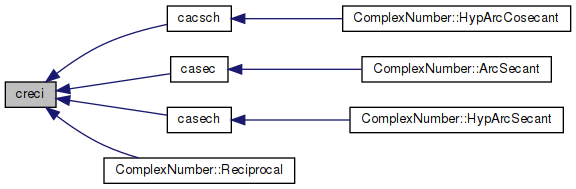## ◆ cround()

 complex cround ( complex z )

Division of two complex numbers.

Definition at line 110 of file prim.c.

References cimag(), cpack(), creal(), and round().

Referenced by ComplexNumber::Round().

111 {
112  complex w;
113  w = cpack(round(creal(z)), round(cimag(z)));
114  return w;
115 }
Definition: mathi.h:48
complex cpack(double x, double y)
Pack two real numbers into a complex number.
Definition: prim.c:68
double cimag(complex z)
Imaginary part of complex number.
Definition: prim.c:46
double creal(complex z)
Real part of complex number.
Definition: prim.c:38
double round(double x)
Round function.
Definition: round.c:40
Here is the call graph for this function: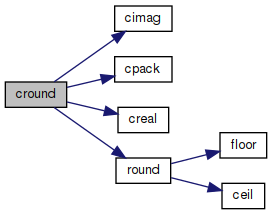Here is the caller graph for this function: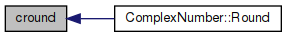## ◆ csec()

 complex csec ( complex z )

Secant of a complex number.

Calculated as in Open Office:
https://wiki.openoffice.org/wiki/Documentation/How_Tos/Calc:_IMSEC_function

```a+bi
2.0 * cos(a) * cosh(b)
real  = ---------------------------—
cosh(2.0 * b) + cos(2.0 * a)```
```            2.0 * sin(a) * sinh(b)
imag  = ---------------------------—
cosh(2.0 * b) + cos(2.0 * a)
```

Definition at line 48 of file csec.c.

References cimag(), cos(), cosh(), cpack(), creal(), sin(), and sinh().

Referenced by ComplexNumber::Secant().

49 {
50  complex w;
51  double a, b;
52  double d;
53
54  a = creal(z);
55  b = cimag(z);
56  d = cosh(2.0 * b) + cos(2.0 * a);
57
58  if (d == 0.0)
59  {
60  w = cpack((double)INFP, (double)INFP);
61  }
62  else
63  {
64  w = cpack((2.0 * cos(a) * cosh(b) / d), (2.0 * sin(a) * sinh(b) / d));
65  }
66
67  return w;
68 }
double sin(double x)
Sine function.
Definition: sin.c:86
double cosh(double x)
Hyperbolic cosine function.
Definition: cosh.c:83
Definition: mathi.h:48
complex cpack(double x, double y)
Pack two real numbers into a complex number.
Definition: prim.c:68
double cimag(complex z)
Imaginary part of complex number.
Definition: prim.c:46
double cos(double x)
Cosine function.
Definition: cos.c:87
#define INFP
Definition: mathr.h:51
double sinh(double x)
Hyperbolic sine function.
Definition: sinh.c:77
double creal(complex z)
Real part of complex number.
Definition: prim.c:38
Here is the call graph for this function: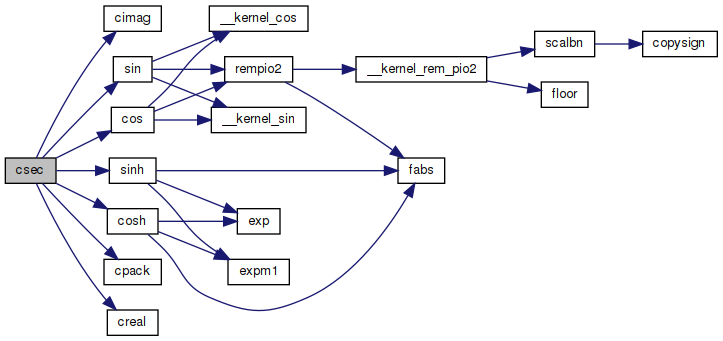Here is the caller graph for this function: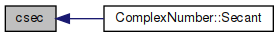## ◆ csech()

 complex csech ( complex z )

Hyperbolic secant of a complex number.

Calculated as in Open Office:
https://wiki.openoffice.org/wiki/Documentation/How_Tos/Calc:_IMSECH_function

```a+bi
2.0 * cosh(a) * cos(b)
real  = ---------------------------—
cosh(2.0 * a) + cos(2.0 * b)```
```        -2.0 * sinh(2.0 * a) * sin(b)
imag  = ---------------------------—
cosh(2.0 * a) + cos(2.0 * b)
```

Definition at line 48 of file csech.c.

References cimag(), cos(), cosh(), cpack(), creal(), sin(), and sinh().

Referenced by ComplexNumber::HypSecant().

49 {
50  complex w;
51  double a, b;
52  double d;
53
54  a = creal(z);
55  b = cimag(z);
56  d = cosh(2.0 * a) + cos(2.0 * b);
57  w = cpack((2.0 * cosh(a) * cos(b) / d), (-2.0 * sinh(a) * sin(b) / d));
58
59  return w;
60 }
double sin(double x)
Sine function.
Definition: sin.c:86
double cosh(double x)
Hyperbolic cosine function.
Definition: cosh.c:83
Definition: mathi.h:48
complex cpack(double x, double y)
Pack two real numbers into a complex number.
Definition: prim.c:68
double cimag(complex z)
Imaginary part of complex number.
Definition: prim.c:46
double cos(double x)
Cosine function.
Definition: cos.c:87
double sinh(double x)
Hyperbolic sine function.
Definition: sinh.c:77
double creal(complex z)
Real part of complex number.
Definition: prim.c:38
Here is the call graph for this function: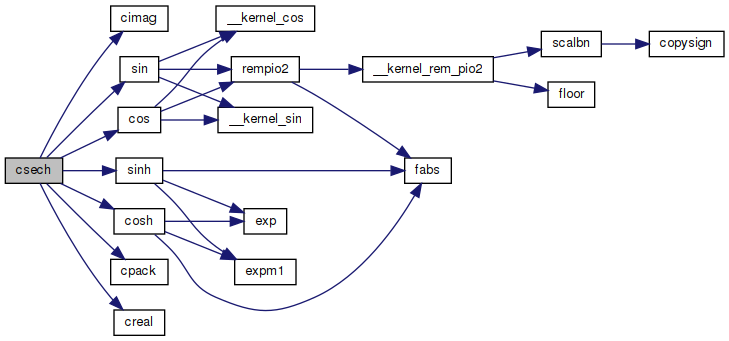Here is the caller graph for this function: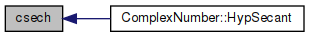## ◆ csgn()

 double csgn ( complex z )

Complex signum.

https://wikipedia.org/wiki/Sign_function#Complex_signum

Definition at line 38 of file csgn.c.

References cimag(), and creal().

Referenced by ComplexNumber::Signum().

39 {
40  double a = creal(z);
41
42  if (a > 0.0)
43  {
44  return 1.0;
45  }
46  else if (a < 0.0)
47  {
48  return -1.0;
49  }
50  else
51  {
52  double b = cimag(z);
53  return b > 0.0 ? 1.0 : b < 0.0 ? -1.0 : 0.0;
54  }
55 }
double cimag(complex z)
Imaginary part of complex number.
Definition: prim.c:46
double creal(complex z)
Real part of complex number.
Definition: prim.c:38
Here is the call graph for this function: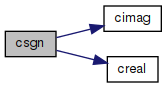Here is the caller graph for this function: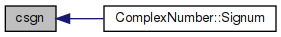## ◆ csin()

 complex csin ( complex z )

Sine of a complex number.

Calculated according to description at wikipedia:
https://wikipedia.org/wiki/Sine#Sine_with_a_complex_argument

```a+bi
real = sin(a) * cosh(b)
imag = cos(a) * sinh(b)
```

Definition at line 50 of file csin.c.

References cchsh(), cimag(), cos(), cpack(), creal(), and sin().

Referenced by ComplexNumber::Sine().

51 {
52  complex w;
53  double a, b;
54  double ch, sh;
55
56  a = creal(z);
57  b = cimag(z);
58  cchsh(b, &ch, &sh);
59  w = cpack((sin(a) * ch), (cos(a) * sh));
60
61  return w;
62 }
double sin(double x)
Sine function.
Definition: sin.c:86
Definition: mathi.h:48
complex cpack(double x, double y)
Pack two real numbers into a complex number.
Definition: prim.c:68
double cimag(complex z)
Imaginary part of complex number.
Definition: prim.c:46
double cos(double x)
Cosine function.
Definition: cos.c:87
void cchsh(double x, double *c, double *s)
Calculate cosh and sinh.
Definition: prim.c:197
double creal(complex z)
Real part of complex number.
Definition: prim.c:38
Here is the call graph for this function: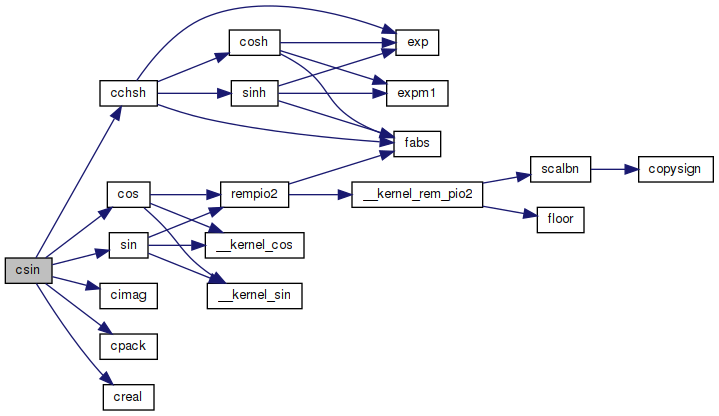Here is the caller graph for this function: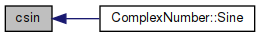## ◆ csinh()

 complex csinh ( complex z )

Hyperbolic sine of a complex number.

Calculated as in Open Office:
https://wiki.openoffice.org/wiki/Documentation/How_Tos/Calc:_IMSINH_function

```a+bi
real = sinh(a) * cos(b)
imag = cosh(a) * sin(b)
```

Definition at line 50 of file csinh.c.

References cchsh(), cimag(), cos(), cpack(), creal(), and sin().

Referenced by ComplexNumber::HypSine().

51 {
52  complex w;
53  double a, b;
54  double ch, sh;
55
56  a = creal(z);
57  b = cimag(z);
58  cchsh(a, &ch, &sh);
59  w = cpack(cos(b) * sh, sin(b) * ch);
60
61  return w;
62 }
double sin(double x)
Sine function.
Definition: sin.c:86
Definition: mathi.h:48
complex cpack(double x, double y)
Pack two real numbers into a complex number.
Definition: prim.c:68
double cimag(complex z)
Imaginary part of complex number.
Definition: prim.c:46
double cos(double x)
Cosine function.
Definition: cos.c:87
void cchsh(double x, double *c, double *s)
Calculate cosh and sinh.
Definition: prim.c:197
double creal(complex z)
Real part of complex number.
Definition: prim.c:38
Here is the call graph for this function: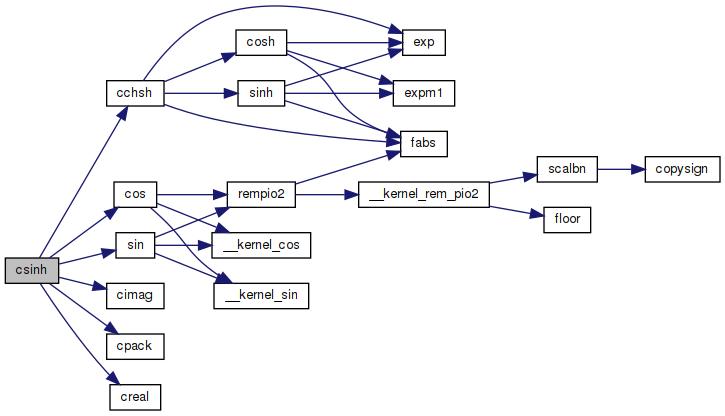Here is the caller graph for this function: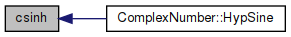## ◆ csqrt()

 complex csqrt ( complex z )

Square root of complex number.

Definition at line 42 of file csqrt.c.

References cabs(), cimag(), cpack(), creal(), fabs(), and sqrt().

Referenced by cacos(), cacosh(), cacsc(), cacsch(), casec(), casech(), casin(), casinh(), and ComplexNumber::SquareRoot().

43 {
44  complex w;
45  double x, y, r, t, scale;
46
47  x = creal(z);
48  y = cimag(z);
49
50  if (y == 0.0)
51  {
52  if (x == 0.0)
53  {
54  w = cpack(0.0, y);
55  }
56  else
57  {
58  r = fabs(x);
59  r = sqrt(r);
60  if (x < 0.0)
61  {
62  w = cpack(0.0, r);
63  }
64  else
65  {
66  w = cpack(r, y);
67  }
68  }
69  return w;
70  }
71  if (x == 0.0)
72  {
73  r = fabs(y);
74  r = sqrt(0.5 * r);
75  if (y > 0)
76  w = cpack(r, r);
77  else
78  w = cpack(r, -r);
79  return w;
80  }
81  /* Rescale to avoid internal overflow or underflow. */
82  if ((fabs(x) > 4.0) || (fabs(y) > 4.0))
83  {
84  x *= 0.25;
85  y *= 0.25;
86  scale = 2.0;
87  }
88  else
89  {
90 #if 1
91  x *= 1.8014398509481984e16; /* 2^54 */
92  y *= 1.8014398509481984e16;
93  scale = 7.450580596923828125e-9; /* 2^-27 */
94 #else
95  x *= 4.0;
96  y *= 4.0;
97  scale = 0.5;
98 #endif
99  }
100  w = cpack(x, y);
101  r = cabs(w);
102  if (x > 0)
103  {
104  t = sqrt(0.5 * r + 0.5 * x);
105  r = scale * fabs((0.5 * y) / t);
106  t *= scale;
107  }
108  else
109  {
110  r = sqrt(0.5 * r - 0.5 * x);
111  t = scale * fabs((0.5 * y) / r);
112  r *= scale;
113  }
114  if (y < 0)
115  w = cpack(t, -r);
116  else
117  w = cpack(t, r);
118  return w;
119 }
Definition: mathi.h:48
complex cpack(double x, double y)
Pack two real numbers into a complex number.
Definition: prim.c:68
double cimag(complex z)
Imaginary part of complex number.
Definition: prim.c:46
double creal(complex z)
Real part of complex number.
Definition: prim.c:38
double sqrt(double x)
Square root function.
Definition: sqrt.c:119
double fabs(double x)
Returns the absolute value of x.
Definition: fabs.c:51
double cabs(complex z)
Absolute value of complex number.
Definition: prim.c:54
Here is the call graph for this function: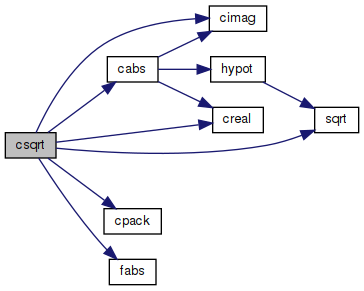Here is the caller graph for this function: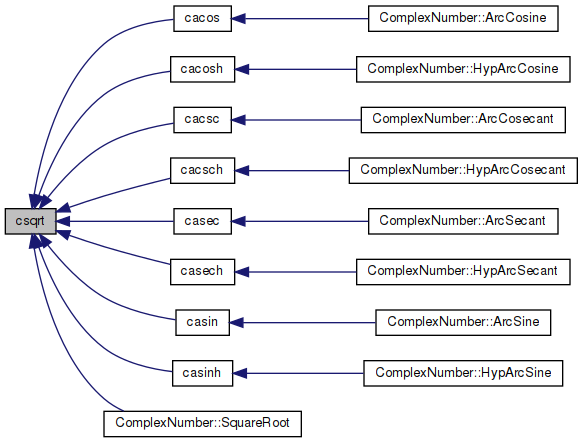## ◆ csub()

 complex csub ( complex a, complex z )

Subtraction of two complex numbers.

Definition at line 130 of file prim.c.

References cimag(), cpack(), and creal().

Referenced by cacos(), cacosh(), cacot(), cacoth(), cacsc(), casec(), casech(), casin(), catan(), catanh(), and ComplexNumber::Sub().

131 {
132  complex w;
133  w = cpack(creal(y) - creal(z), cimag(y) - cimag(z));
134  return w;
135 }
Definition: mathi.h:48
complex cpack(double x, double y)
Pack two real numbers into a complex number.
Definition: prim.c:68
double cimag(complex z)
Imaginary part of complex number.
Definition: prim.c:46
double creal(complex z)
Real part of complex number.
Definition: prim.c:38
Here is the call graph for this function: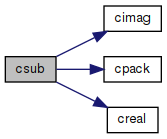Here is the caller graph for this function: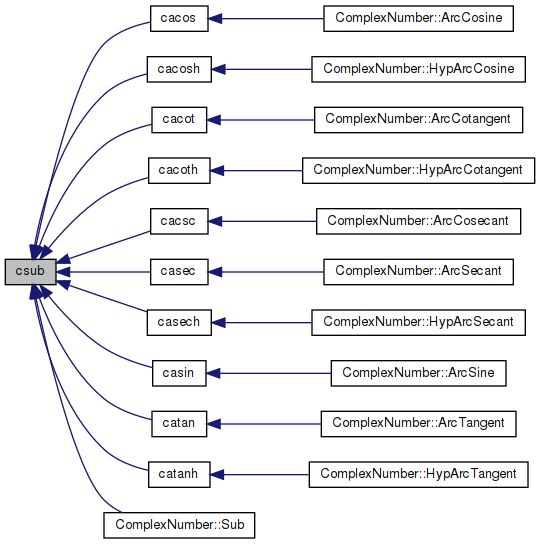## ◆ ctan()

 complex ctan ( complex z )

Tangent of a complex number.

Calculated as in Open Office:
https://wiki.openoffice.org/wiki/Documentation/How_Tos/Calc:_IMTAN_function

```a+bi
sin(2.0 * a)
real  = ---------------------------—
cos(2.0 * a) + cosh(2.0 * b)
sinh(2.0 * b)

imag  = ---------------------------—
cos(2.0 * a) + cosh(2.0 * b)
```

Definition at line 55 of file ctan.c.

References cimag(), cos(), cosh(), cpack(), creal(), sin(), and sinh().

Referenced by ComplexNumber::Tangent().

56 {
57  complex w;
58  double a, b;
59  double d;
60
61  a = creal(z);
62  b = cimag(z);
63  d = cos(2.0 * a) + cosh(2.0 * b);
64
65  if (d == 0.0)
66  {
67  w = cpack((double)INFP, (double)INFP);
68  }
69  else
70  {
71  w = cpack((sin(2.0 * a) / d), (sinh(2.0 * b) / d));
72  }
73
74  return w;
75 }
double sin(double x)
Sine function.
Definition: sin.c:86
double cosh(double x)
Hyperbolic cosine function.
Definition: cosh.c:83
Definition: mathi.h:48
complex cpack(double x, double y)
Pack two real numbers into a complex number.
Definition: prim.c:68
double cimag(complex z)
Imaginary part of complex number.
Definition: prim.c:46
double cos(double x)
Cosine function.
Definition: cos.c:87
#define INFP
Definition: mathr.h:51
double sinh(double x)
Hyperbolic sine function.
Definition: sinh.c:77
double creal(complex z)
Real part of complex number.
Definition: prim.c:38
Here is the call graph for this function: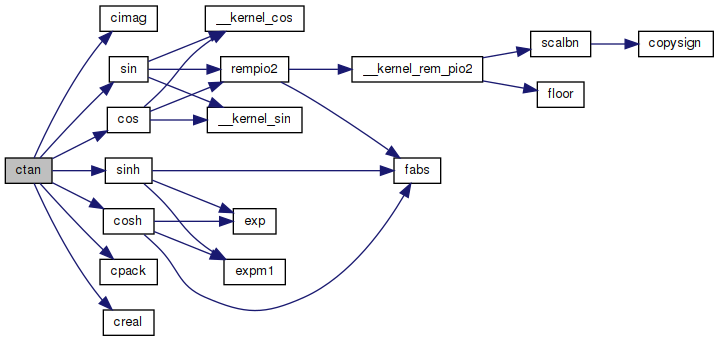Here is the caller graph for this function: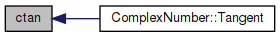## ◆ ctanh()

 complex ctanh ( complex z )

Hyperbolic tangent of a complex number.

```a+bi
sinh(2.0 * a)
real  = ---------------------------—
cosh(2.0 * a) + cos(2.0 * b)
sin(2.0 * b)

imag  = ---------------------------—
cosh(2.0 * a) + cos(2.0 * b)
```

Definition at line 53 of file ctanh.c.

References cimag(), cos(), cosh(), cpack(), creal(), sin(), and sinh().

Referenced by ComplexNumber::HypTangent().

54 {
55  complex w;
56  double a, b;
57  double d;
58
59  a = creal(z);
60  b = cimag(z);
61  d = cosh(2.0 * a) + cos(2.0 * b);
62  w = cpack((sinh(2.0 * a) / d), (sin(2.0 * b) / d));
63
64  return w;
65 }
double sin(double x)
Sine function.
Definition: sin.c:86
double cosh(double x)
Hyperbolic cosine function.
Definition: cosh.c:83
Definition: mathi.h:48
complex cpack(double x, double y)
Pack two real numbers into a complex number.
Definition: prim.c:68
double cimag(complex z)
Imaginary part of complex number.
Definition: prim.c:46
double cos(double x)
Cosine function.
Definition: cos.c:87
double sinh(double x)
Hyperbolic sine function.
Definition: sinh.c:77
double creal(complex z)
Real part of complex number.
Definition: prim.c:38
Here is the call graph for this function: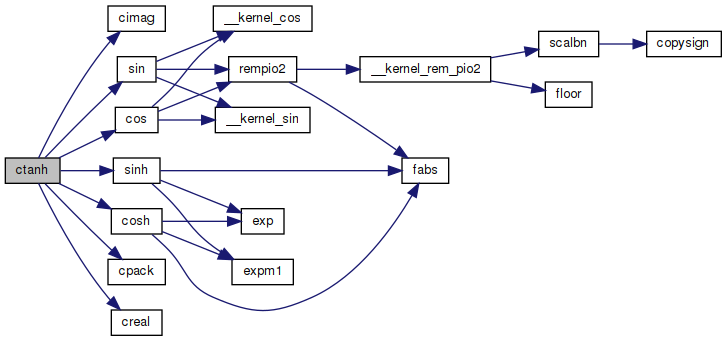Here is the caller graph for this function: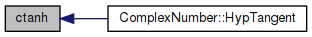## ◆ ctrunc()

 complex ctrunc ( complex z )

Truncated value of complex number.

Definition at line 80 of file prim.c.

References cimag(), cpack(), creal(), and trunc().

Referenced by ComplexNumber::Trunc().

81 {
82  complex w;
83  w = cpack(trunc(creal(z)), trunc(cimag(z)));
84  return w;
85 }
Definition: mathi.h:48
complex cpack(double x, double y)
Pack two real numbers into a complex number.
Definition: prim.c:68
double cimag(complex z)
Imaginary part of complex number.
Definition: prim.c:46
double creal(complex z)
Real part of complex number.
Definition: prim.c:38
double trunc(double x)
Truncate function.
Definition: trunc.c:52
Here is the call graph for this function: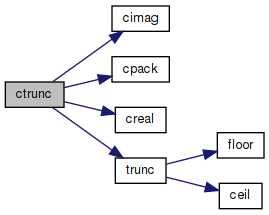Here is the caller graph for this function: